• 优化求解】基于matlab模拟退火算法求解函数极值问题【含Matlab源码 1203期】
2021-08-09 20:19:48

## 一、获取代码方式

获取代码方式1：
通过订阅紫极神光博客付费专栏，凭支付凭证，私信博主，可获得此代码。

获取代码方式2：
完整代码已上传我的资源：【优化求解】基于matlab模拟退火算法求解函数极值问题【含Matlab源码 1203期】

备注：
订阅紫极神光博客付费专栏，可免费获得2份代码（有效期为订阅日起，三天内有效）；

## 二、模拟退火算法简介

1 引言
模拟退火算法(Simulated Annealing，SA)的思想最早由Metropolis等人于1953年提出：Kirkpatrick于1983年第一次使用模拟退火算法求解组合最优化问题 。模拟退火算法是一种基于MonteCarlo迭代求解策略的随机寻优算法， 其出发点是基于物理中固体物质的退火过程与一般组合优化问题之间的相似性。其目的在于为具有NP(Non-deterministic Polynomial) 复杂性的问题提供有效的近似求解算法，它克服了其他优化过程容易陷入局部极小的缺陷和对初值的依赖性。
模拟退火算法是一种通用的优化算法，是局部搜索算法的扩展。它不同于局部搜索算法之处是以一定的概率选择邻域中目标值大的劣质解。从理论上说，它是一种全局最优算法。模拟退火算法以优化问
题的求解与物理系统退火过程的相似性为基础， 它利用Metropolis算法并适当地控制温度的下降过程来实现模拟退火，从而达到求解全局优化问题的目的.
模拟退火算法是一种能应用到求最小值问题的优化过程。在此过程中，每一步更新过程的长度都与相应的参数成正比，这些参数扮演着温度的角色。与金属退火原理相类似，在开始阶段为了更快地最小
化，温度被升得很高，然后才慢慢降温以求稳定。
目前，模拟退火算法迎来了兴盛时期，无论是理论研究还是应用研究都成了十分热门的课题[3-7]。尤其是它的应用研究显得格外活跃，已在工程中得到了广泛应用，诸如生产调度、控制工程、机器学习、神经网络、模式识别、图像处理、离散/连续变量的结构优化问题等领域。它能有效地求解常规优化方法难以解决的组合优化问题和复杂函数优化问题，适用范围极广。
模拟退火算法具有十分强大的全局搜索性能，这是因为比起普通的优化搜方法，它采用了许多独特的方法和技术：在模拟退火算法中，基本不用搜索空间的知识或者其他的辅助信息，而只是定义邻域
结构，在其邻域结构内选取相邻解，再利用目标函数进行评估：模拟退火算法不是采用确定性规则，而是采用概率的变迁来指导它的搜索方向，它所采用的概率仅仅是作为一种工具来引导其搜索过程朝着更优化解的区域移动。因此，虽然看起来它是一种盲目的搜索方法，但实际上有着明确的搜索方向。

2 模拟退火算法理论
模拟退火算法以优化问题求解过程与物理退火过程之间的相似性为基础，优化的目标函数相当于金属的内能，优化问题的自变量组合状态空间相当于金属的内能状态空间，问题的求解过程就是找一个组
合状态， 使目标函数值最小。利用Me to polis算法并适当地控制温度的下降过程实现模拟退火，从而达到求解全局优化问题的目的.
2.1物理退火过程
模拟退火算法的核心思想与热力学的原理极为类似。在高温下，液体的大量分子彼此之间进行着相对自由移动。如果该液体慢慢地冷却，热能原子可动性就会消失。大量原子常常能够自行排列成行，形成一个纯净的晶体，该晶体在各个方向上都被完全有序地排列在几百万倍于单个原子的距离之内。对于这个系统来说，晶体状态是能量最低状态，而所有缓慢冷却的系统都可以自然达到这个最低能量状态。实际上，如果液态金属被迅速冷却，则它不会达到这一状态，而只能达到一种只有较高能量的多晶体状态或非结晶状态。因此，这一过程的本质在于缓慢地冷却，以争取足够的时间，让大量原子在丧失可动性之前进行重新分布，这是确保能量达到低能量状态所必需的条件。简单而言，物理退火过程由以下几部分组成：加温过程、等温过程和冷却过程。

加温过程
其目的是增强粒子的热运动，使其偏离平衡位置。当温度足够高时，固体将熔解为液体，从而消除系统原先可能存在的非均匀态，使随后进行的冷却过程以某一平衡态为起点。熔解过程与系统的能量增
大过程相联系，系统能量也随温度的升高而增大。

等温过程
通过物理学的知识得知，对于与周围环境交换热量而温度不变的封闭系统，系统状态的自发变化总是朝着自由能减小的方向进行：当自由能达到最小时，系统达到平衡态。

冷却过程
其目的是使粒子的热运动减弱并逐渐趋于有序，系统能量逐渐下降，从而得到低能量的晶体结构。

2.2 模拟退火原理
模拟退火算法来源于固体退火原理，将固体加温至充分高，再让其徐徐冷却。加温时，固体内部粒子随温升变为无序状，内能增大：而徐徐冷却时粒子渐趋有序，在每个温度上都达到平衡态，最后在常
温时达到基态，内能减为最小。模拟退火算法与金属退火过程的相似关系如表7.1所示。根Metropolis准则， 粒子在温度7时趋于平衡的概率为exp(-▲E/T) ， 其中E为温度7时的内能， ▲E为其改变量。用
固体退火模拟组合优化问题，将内能E模拟为目标函数值，温度7演化成控制参数，即得到解组合优化问题的模拟退火算法：由初始解%和控制参数初值7开始，对当前解重复“产生新解→计算目标函数差一接受或舍弃”的迭代，并逐步减小T值，算法终止时的当前解即为所得近似最优解， 这是基MonteCarlo迭代求解法的一种启发式随机搜索过程。退火过程由冷却进度表控制，包括控制参数的初值7及其衰
减因子K、每个7值时的迭代次数I和停止条件。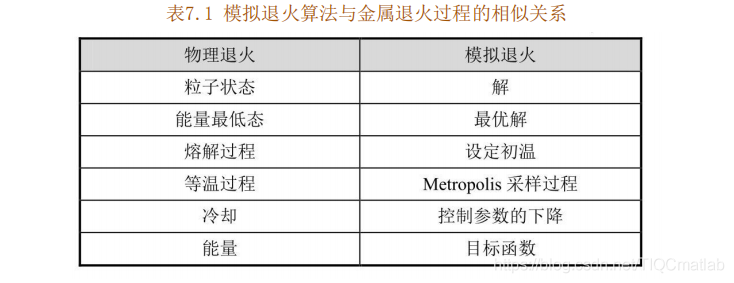2.3 模拟退火算法思想
模拟退火的主要思想是：在搜索区间随机游走(即随机选择点)，再利用Metropolis抽样准则， 使随机游走逐渐收敛于局部最优解。而温度是Metropolis算法中的一个重要控制参数，可以认为这个参数的大小控制了随机过程向局部或全局最优解移动的快慢。Metropolis是一种有效的重点抽样法， 其算法为：系统从一个能量状态变化到另一个状态时，相应的能量从E变化到E，其概率为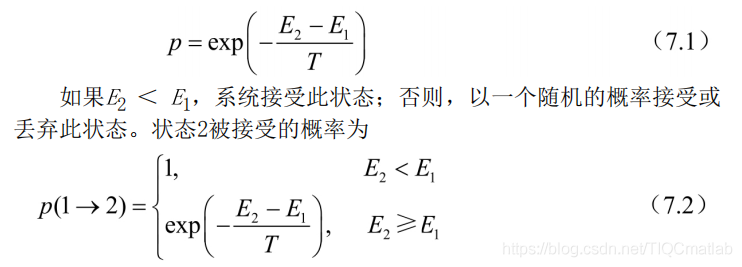这样经过一定次数的迭代，系统会逐渐趋于一个稳定的分布状态。
重点抽样时，新状态下如果向下，则接受(局部最优)；若向上(全局搜索)，则以一定概率接受。模拟退火算法从某个初始解出发，经过大量解的变换后，可以求得给定控制参数值时组合优化问题的相对最优解。然后减小控制参数7的值， 重复执行Metropolis算法，就可以在控制参数T趋于零时，最终求得组合优化问题的整体最优解。控制参数的值必须缓慢衰减。温度是Metropolis算法的一个重要控制参数， 模拟退火可视为递减控制参数7时Metro pl is算法的迭代。开始时7值大， 可以接受较差的恶化解；随着7的减小，只能接受较好的恶化解；最后在7趋于0时，就不再接受任何恶化解了。
在无限高温时，系统立即均匀分布，接受所有提出的变换。T的衰减越小， 7到达终点的时间越长； 但可使马尔可夫(Markov) 链减小，以使到达准平衡分布的时间变短。

2.4 模拟退火算法的特点
模拟退火算法适用范围广，求得全局最优解的可靠性高，算法简单，便于实现；该算法的搜索策略有利于避免搜索过程因陷入局部最优解的缺陷，有利于提高求得全局最优解的可靠性。模拟退火算法具
有十分强的鲁棒性，这是因为比起普通的优化搜索方法，它采用许多独特的方法和技术。主要有以下几个方面：
(1)以一定的概率接受恶化解。
模拟退火算法在搜索策略上不仅引入了适当的随机因素，而且还引入了物理系统退火过程的自然机理。这种自然机理的引入，使模拟退火算法在迭代过程中不仅接受使目标函数值变“好”的点，而且还能够以一定的概率接受使目标函数值变“差”的点。迭代过程中出现的状态是随机产生的，并且不强求后一状态一定优于前一状态，接受概率随着温度的下降而逐渐减小。很多传统的优化算法往往是确定性
的，从一个搜索点到另一个搜索点的转移有确定的转移方法和转移关系，这种确定性往往可能使得搜索点远达不到最优点，因而限制了算法的应用范围。而模拟退火算法以一种概率的方式来进行搜索，增加了搜索过程的灵活性。
(2)引进算法控制参数。
引进类似于退火温度的算法控制参数，它将优化过程分成若干阶段， 并决定各个阶段下随机状态的取舍标准， 接受函数由Metropolis算法给出一个简单的数学模型。模拟退火算法有两个重要的步骤：一
是在每个控制参数下，由前迭代点出发，产生邻近的随机状态，由控制参数确定的接受准则决定此新状态的取舍，并由此形成一定长度的随机Markov链； 二是缓慢降低控制参数， 提高接受准则， 直至控制参数趋于零，状态链稳定于优化问题的最优状态，从而提高模拟退火算法全局最优解的可靠性。
(3)对目标函数要求少。
传统搜索算法不仅需要利用目标函数值，而且往往需要目标函数的导数值等其他一些辅助信息才能确定搜索方向：当这些信息不存在时，算法就失效了。而模拟退火算法不需要其他的辅助信息，而只是
定义邻域结构，在其邻域结构内选取相邻解，再用目标函数进行评估。

2.5模拟退火算法的改进方向
在确保一定要求的优化质量基础上，提高模拟退火算法的搜索效率，是对模拟退火算法改进的主要内容[9-10]。有如下可行的方案：选择合适的初始状态；设计合适的状态产生函数，使其根据搜索进程的
需要表现出状态的全空间分散性或局部区域性：设计高效的退火过程；改进对温度的控制方式：采用并行搜索结构；设计合适的算法终止准则：等等。
此外，对模拟退火算法的改进，也可通过增加某些环节来实现。
主要的改进方式有：
(1)增加记忆功能。为避免搜索过程中由于执行概率接受环节而遗失当前遇到的最优解，可通过增加存储环节，将到目前为止的最好状态存储下来。
(2)增加升温或重升温过程。在算法进程的适当时机，将温度适当提高，从而可激活各状态的接受概率，以调整搜索进程中的当前状态，避兔算法在局部极小解处停滞不前。
(3)对每一当前状态，采用多次搜索策略，以概率接受区域内的最优状态，而不是标准模拟退火算法的单次比较方式。
(4)与其他搜索机制的算法(如遗传算法、免疫算法等)相结合。可以综合其他算法的优点，提高运行效率和求解质量。

3 模拟退火算法流程
模拟退火算法新解的产生和接受可分为如下三个步骤：
(1)由一个产生函数从当前解产生一个位于解空间的新解；为便于后续的计算和接受，减少算法耗时，通常选择由当前解经过简单变换即可产生新解的方法。注意，产生新解的变换方法决定了当前新解
的邻域结构，因而对冷却进度表的选取有一定的影响。
(2)判断新解是否被接受，判断的依据是一个接受准则，最常用的接受准则是Metropolis准则：若AK 0， 则接受X作为新的当前解否则， 以概率exp(-▲E/7) 接受X”作为新的当前解X.
(3)当新解被确定接受时，用新解代替当前解，这只需将当前解中对应于产生新解时的变换部分予以实现，同时修正目标函数值即可。此时，当前解实现了一次迭代，可在此基础上开始下一轮试验。若当新解被判定为舍弃，则在原当前解的基础上继续下一轮试验。模拟退火算法求得的解与初始解状态(算法迭代的起点)无关，具有渐近收敛性，已在理论上被证明是一种以概率1收敛于全局最优解的优化算法。模拟退火算法可以分解为解空间、目标函数和初始解三部分。该算法具体流程如下：
(1)初始化：设置初始温度7(充分大)、初始解状态%(是算
法迭代的起点)、每个7值的迭代次数L：
(2)对k=1，…，L做第(3)至第(6)步；
(3)产生新解X；
(4) 计算增量A BE(X) -E(X) ， 其中E() ) 为评价函数：
(5)若AK0，则接受X作为新的当前解，否则以概率
exp(-▲E/7) 接受X作为新的当前解；
(6)如果满足终止条件，则输出当前解作为最优解，结束程序：
(7)7逐渐减小，且T→0，然后转第(2)步。
模拟退火算法流程如图7.1所示。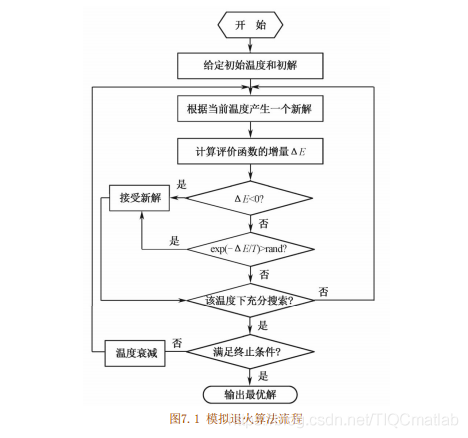4 关键参数说明
模拟退火算法的性能质量高，它比较通用，而且容易实现。不过，为了得到最优解，该算法通常要求较高的初温以及足够多次的抽样，这使算法的优化时间往往过长。从算法结构知，新的状态产生函
数、初温、退温函数、Markov链长度和算法停止准则， 是直接影响算法优化结果的主要环节。
状态产生函数
设计状态产生函数应该考虑到尽可能地保证所产生的候选解遍布全部解空间。一般情况下状态产生函数由两部分组成，即产生候选解的方式和产生候选解的概率分布。候选解的产生方式由问题的性质决
定，通常在当前状态的邻域结构内以一定概率产生。
初温
温度7在算法中具有决定性的作用，它直接控制着退火的走向。由随机移动的接受准则可知：初温越大，获得高质量解的几率就越大，且Metropolis的接收率约为1。然而， 初温过高会使计算时间增加。
为此，可以均匀抽样一组状态，以各状态目标值的方差为初温。
退温函数
退温函数即温度更新函数，用于在外循环中修改温度值。目前，最常用的温度更新函数为指数退温函数， 即T(n+1) =KXT(n) ， 其中0<K1是一个非常接近于1的常数。
Markov链长度L的选取
Markov链长度是在等温条件下进行迭代优化的次数， 其选取原则是在衰减参数7的衰减函数己选定的前提下，L应选得在控制参数的每一取值上都能恢复准平衡，一般L取100~1000.
算法停止准则
算法停止准则用于决定算法何时结束。可以简单地设置温度终值T，当F=T，时算法终止。然而，模拟火算法的收敛性理论中要求T趋向于零，这其实是不实际的。常用的停止准则包：设置终止温度的阈
值，设置迭代次数阈值，或者当搜索到的最优值连续保持不变时停止搜索。

## 三、案例及完整源代码

1 案例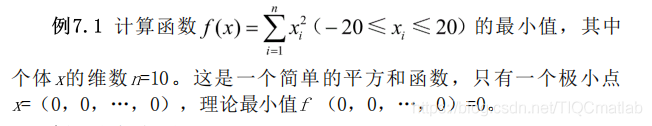2 完整代码

%%%%%%%%%%%%%%%%%%%%%%模拟退火算法解决函数极值%%%%%%%%%%%%%%%%%%%%%%
%%%%%%%%%%%%%%%%%%%%%%%%%%%%%初始化%%%%%%%%%%%%%%%%%%%%%%%%%%%%%%%%
clear all;                      %清除所有变量
close all;                      %清图
clc;                            %清屏
D=10;                           %变量维数
Xs=20;                          %上限
Xx=-20;                         %下限
%%%%%%%%%%%%%%%%%%%%%%%%%%%冷却表参数%%%%%%%%%%%%%%%%%%%%%%%%%%%%%%
L = 200;                        %马可夫链长度
K = 0.998;                      %衰减参数
S = 0.01;                       %步长因子
T=100;                          %初始温度
YZ = 1e-8;                      %容差
P = 0;                          %Metropolis过程中总接受点
%%%%%%%%%%%%%%%%%%%%%%%%%%随机选点 初值设定%%%%%%%%%%%%%%%%%%%%%%%%%
PreX = rand(D,1)*(Xs-Xx)+Xx;
PreBestX = PreX;
PreX =  rand(D,1)*(Xs-Xx)+Xx;
BestX = PreX;
%%%%%%%%%%%每迭代一次退火一次(降温), 直到满足迭代条件为止%%%%%%%%%%%%
deta=abs( func1( BestX)-func1(PreBestX));
while (deta > YZ) && (T>0.001)
T=K*T;
%%%%%%%%%%%%%%%%%%%%%在当前温度T下迭代次数%%%%%%%%%%%%%%%%%%%%%%
for i=1:L
%%%%%%%%%%%%%%%%%在此点附近随机选下一点%%%%%%%%%%%%%%%%%%%%%
NextX = PreX + S* (rand(D,1) *(Xs-Xx)+Xx);
%%%%%%%%%%%%%%%%%边界条件处理%%%%%%%%%%%%%%%%%%%%%%%%%%
for ii=1:D
if NextX(ii)>Xs | NextX(ii)<Xx
NextX(ii)=PreX(ii) + S* (rand *(Xs-Xx)+Xx);
end
end
%%%%%%%%%%%%%%%%%%%%%%%是否全局最优解%%%%%%%%%%%%%%%%%%%%%%
if (func1(BestX) > func1(NextX))
%%%%%%%%%%%%%%%%%%保留上一个最优解%%%%%%%%%%%%%%%%%%%%%
PreBestX = BestX;
%%%%%%%%%%%%%%%%%%%此为新的最优解%%%%%%%%%%%%%%%%%%%%%%
BestX=NextX;
end
%%%%%%%%%%%%%%%%%%%%%%%% Metropolis过程%%%%%%%%%%%%%%%%%%%
if( func1(PreX) - func1(NextX) > 0 )
%%%%%%%%%%%%%%%%%%%%%%%接受新解%%%%%%%%%%%%%%%%%%%%%%%%
PreX=NextX;
P=P+1;
else
changer = -1*(func1(NextX)-func1(PreX))/ T ;
p1=exp(changer);
%%%%%%%%%%%%%%%%%%%%%%%%接受较差的解%%%%%%%%%%%%%%%%%%%%
if p1 > rand
PreX=NextX;
P=P+1;
end
end
trace(P+1)=func1( BestX);
end
deta=abs( func1( BestX)-func1 (PreBestX));
end
disp('最小值在点:');
BestX
disp( '最小值为:');
func1(BestX)
figure
plot(trace(2:end))
xlabel('迭代次数')
ylabel('目标函数值')
title('适应度进化曲线')


%%%%%%%%%%%%%%%%%%%%%%%适应度函数%%%%%%%%%%%%%%%%%%%%%%%%
function result=func1(x)
summ=sum(x.^2);
result=summ;


## 四、解题过程及运行结果

1 解题过程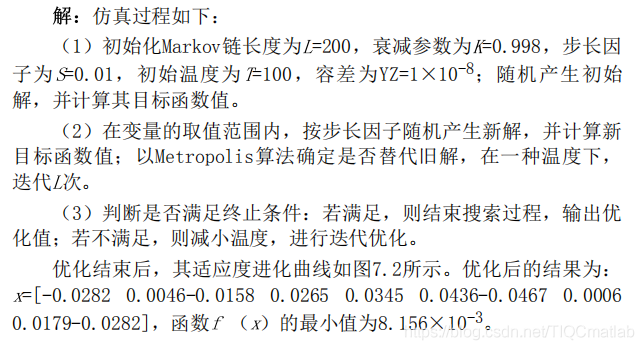2 运行结果## 五、matlab版本及参考文献

1 matlab版本
2014a

2 参考文献
 包子阳,余继周,杨杉.智能优化算法及其MATLAB实例（第2版）[M].电子工业出版社，2016.
张岩,吴水根.MATLAB优化算法源代码[M].清华大学出版社，2017.matlab
更多相关内容

遗传算法是群智能算法中的一个分支，是一类基于种群搜索的优化算法，受自然界生物进化机制的启发，通过自然选择、变异、重组等操作，针对特定的问题取寻找出一个满意的解。其遗传进化过程简单，容易理解，是其他一些遗传算法的基础。
遗传算法的搜索特点是以编码空间代替问题的参数空间，以适应度函数为评价依据；将编码集作为遗传的基础，对个体进行遗传操作，建立一个迭代过程。首先，算法从一个由个体组成的种群开始，对每个种群个体进行适应度评价；其次，利用个体的适应度选择个体，并利用交叉和变异等遗传算子作用其上，产生后代个体；最后，在原种群个体和后代个体中选择个体生成下一代种群。
本文主要通过遗传算法来求解函数优化和TSP两个问题。函数优化是我们随时都是遇到的问题，而传统的求解方法更多的是基于数学理论来求解，通过求导等一系列数学计算来发掘函数本身的单调性等一系列性质并以此来寻找函数的极大极小值，但是普通的求解方法对问题本身要求很高，例如函数必须可导，或者必须是凸函数等，所以在面对一些非凸函数或者奇异函数时就不可以用传统求解方法，而只能采用暴力搜索等算法。但是遗传算法对于函数本身没有要求，它可以看作是一种指导性搜索算法，利用前一代所遗留的信息来指导下一代的进化，这样每一个计算得到的值都可以在此利用，不重复计算。所以遗传算法在求解一些复杂函数最优值的问题中被广泛使用。
TSP问题是一个典型的NP问题，暴力求解的复杂度非常高，但是遗传算法的提出可以使得其在可接受迭代次数内达到收敛，本文利用遗传算法来求解所给城市的最优路线。

## 一、问题重述

根据遗传算法求解问题，分别运用遗传算法求解低维单目标优化问题，高维单目标优化问题和TSP问题。

## 二、背景和发展

遗传算法是近年来迅速发展起来的一种全新的随机搜索与优化算法，其基本思想是基于达尔文的遗传学说，该算法于1975年创建。
1971年，首次将遗传算法用于函数优化。
1975年，出版了《自然系统和人工系统的自适应》，第一本系统的阐述了遗传算法的著作。
1989年，《搜索，优化和机器学习中的遗传算法》，总结了遗传算法研究的主要成果，对遗传算法及其应用做了全面而系统的阐述，同年并提出了基于自然选择远测创造性的提出了用层次化的计算机程序来表达问题的遗传程序设计方法。
1991年，提出了基于邻域交叉的交叉算子，并将其运用到TSP问题中。
目前，遗传算法已经广泛应用，主要有基于遗传算法的机器学习，遗传算法和神经网络，并行处理人工生命等领域。

## 三、原理分析

### 3.1 定义

进化计算的基本思想来源于生物学中的基本知识：生物从简单到复杂，从低级到高级的进化过程是一个自然的稳健的优化过程。这一进化过程的目的在于使生命个体更好的适应周边环境。生物种群通过“优胜劣汰”及遗传变异来达到进化的目的。
目前研究的进化算法主要有四种：遗传算法，进化规划，进化策略和遗传编程。
遗传算法（Genetic Algorithm）是模拟达尔文生物进化论的自然选择和遗传学机理的生物进化过程的计算模型，包括选择、交叉和变异操作。
标准的遗传算法主要有如下主要特点：
1，遗传算法必须通过适当的方法对问题的可行解进行编码。解空间中的可行解是个体的表现型，它在遗传算法的搜索空间中对应的编码形式是个体的基因型。
2，遗传算法基于个体的适应度来进行概率选择操作。
3，在遗传算法中，个体的重组使用交叉算子。交叉算子是遗传算法所强调的关键技术，它是遗传算法中产生个体的主要方法，也是遗传算法区别于其他进化算法的一个主要特点。
4，在遗传算法中，编译操作使用随机变异技术。
5，遗传算法擅长对离散空间的搜索，它较多地应用于组合优化问题。
直接对结构对象进行操作，不存在求导和函数连续性的限定；具有内在的隐并行性和更好的全局寻优能力；采用概率化的寻优方法，不需要确定的规则就能自动获取和指导优化的搜索空间，自适应地调整搜索方向。
遗传算法以一种群体中的所有个体为对象，并利用随机化技术指导对一个被编码的参数空间进行高效搜索。其中，选择、交叉和变异构成了遗传算法的遗传操作；参数编码、初始群体的设定、适应度函数的设计、遗传操作设计、控制参数设定五个要素组成了遗传算法的核心内容。
相关术语：
• 基因型(genotype)：性状染色体的内部表现；
• 表现型(phenotype)：染色体决定的性状的外部表现，或者说，根据基因型形成的个体的外部表现；
• 进化(evolution)：种群逐渐适应生存环境，品质不断得到改良。生物的进化是以种群的形式进行的。
• 适应度(fitness)：度量某个物种对于生存环境的适应程度。
• 选择(selection)：以一定的概率从种群中选择若干个个体。一般，选择过程是一种基于适应度的优胜劣汰的过程。
• 复制(reproduction)：细胞分裂时，遗传物质DNA通过复制而转移到新产生的细胞中，新细胞就继承了旧细胞的基因。
• 交叉(crossover)：两个染色体的某一相同位置处DNA被切断，前后两串分别交叉组合形成两个新的染色体。也称基因重组或杂交；
• 变异(mutation)：复制时可能（很小的概率）产生某些复制差错，变异产生新的染色体，表现出新的性状。
• 编码(coding)：DNA中遗传信息在一个长链上按一定的模式排列。遗传编码可看作从表现型到基因型的映射。
• 解码(decoding)：基因型到表现型的映射。
• 个体（individual）：指染色体带有特征的实体；
• 种群（population）：个体的集合，该集合内个体数称为种群。

### 3.2 遗传算法的基本流程

遗传算法的搜索特点是以编码空间代替问题的参数空间，以适应度函数为评价依据；将编码集作为遗传的基础，对个体进行遗传操作，建立一个迭代过程。首先，算法从一个由个体组成的种群开始，对每个种群个体进行适应度评价；其次，利用个体的适应度选择个体，并利用交叉和变异等遗传算子作用其上，产生后代个体；最后，在原种群个体和后代个体中选择个体生成下一代种群。### 3.3 编码

染色体作为遗传物质的主要载体，即多个基因的集合，其内部表现（即基因型）是某种基因组合，它决定了个体的形状的外部表现，如黑头发的特征是由染色体中控制这一特征的某种基因组合决定的。因此，在一开始需要实现从表现型到基因型的映射即编码工作。
遗传算法的编码有浮点编码、二进制编码和符号编码，这里只介绍二进制编码规则。二进制编码既符合计算机处理信息的原理，也方便了对染色体进行遗传、编译和突变等操作。设某一参数的取值范围为（L，U），使用长度为k的二进制编码表示该参数，则它共有2^k种不同的编码。该参数编码时的对应关系为易知其取值精度δ满足关系：

δ = ( U − L ) / ( 2 k − 1 ) δ=(U-L)/(2^k-1)
假设用长度为k的二进制编码表示该参数，编码为 x : b k b ( k − 1 ) b ( k − 2 ) b ( k − 3 ) … b 2 b 1 x:b_k b_(k-1) b_(k-2) b_(k-3)…b_2 b_1 ，对应的解码公式为：### 3.4 适应度函数

为了体现染色体的适应能力，区分种群中个体的好坏，遗传算法引入了对问题中的每个染色体都能进行度量的函数，即适应度函数。通过适应度函数来决定染色体的优劣程度，它体现了自然进化中的优胜劣汰原则。在简单问题的优化时，通常可以直接将目标函数换成适应度函数。在复杂问题的优化时，往往需要构造合适的评价函数，使其适应遗传算法进行优化。好的适应度函数能够真实反映优化的情况，找到问题真正的最优解，质量差的适应度函数可能是的优化后的解不可用。

### 3.5选择算子

选择算子体现了自然界中优胜劣汰的基本规律。个体的适应度值所度量的优劣程度决定了它在下一代是被淘汰还是被遗传，从而提高全局收敛性和计算效率。一般来说，如果该个体适应度函数值比较大，则它存在的概率也比较大。
通常采用的选择方法有：轮盘赌选择，竞争选择，随机遍历抽样选择，竞标赛选择等。其中最知名的为轮盘赌选择，其基本原理是：首先计算种群中个体的适应度值，然后计算该个体的适应度值在该种群中所占的比例，该比例就为该个体的选择概率或生存概率。个体 x i x_i 的选择概率如下：其中， f ( x i ) f(x_i) 为个体的适应度值，N为种群的规模大小。根据这个概率分布选取N个个体产生下一代种群。

### 3.6 交叉算子

遗传算法的交叉操作，是指对两个相互配对的染色体按某种方式相互交换其部分基因，从而形成两个新的个体。
交叉算子体现了信息交换的思想。交叉又称为重组，是按较大的概率从种群中选择两个个体，交换两个个体的某个或某些位。其作用是组合出新的个体，在编码串空间进行有效搜索，同时降低对有效模式的破坏概率。交叉算子的设计包括如何确定交叉点的位置和如何交换两个方面，但在设计交叉算子时，需要保证前一代中有优秀个体的性状能够在后一代的新个体中尽可能得到遗传和继承。个体采用二进制编码时，常用的交叉算子有单点交叉，两点交叉和均匀交叉。
1，单点交叉（One-point Crossover）：指在个体编码串中只随机设置一个交叉点，然后再该点相互交换两个配对个体的部分染色体。
2，两点交叉与多点交叉：
两点交叉（Two-point Crossover）：在个体编码串中随机设置了两个交叉点，然后再进行部分基因交换。
多点交叉（Multi-point Crossover）
3，均匀交叉（也称一致交叉，Uniform Crossover）：两个配对个体的每个基因座上的基因都以相同的交叉概率进行交换，从而形成两个新个体。### 3.7 变异算子

变异算子是指从种群中随机选择一个个体，以变异概率对个体编码串上的某个或某些位置进行改变，经过变异后形成一个新的染色体。在遗传算法中，能够保持种群多样性的一个主要途径就是通过个体变异。
• 基本位变异（Simple Mutation）：对个体编码串中以变异概率、随机指定的某一位或某几位仅因座上的值做变异运算。
• 均匀变异（Uniform Mutation）：分别用符合某一范围内均匀分布的随机数，以某一较小的概率来替换个体编码串中各个基因座上的原有基因值。（特别适用于在算法的初级运行阶段）
• 边界变异（Boundary Mutation）：随机的取基因座上的两个对应边界基因值之一去替代原有基因值。特别适用于最优点位于或接近于可行解的边界时的一类问题。
• 非均匀变异：对原有的基因值做一随机扰动，以扰动后的结果作为变异后的新基因值。对每个基因座都以相同的概率进行变异运算之后，相当于整个解向量在解空间中作了一次轻微的变动。
• 高斯近似变异：进行变异操作时用符号均值为Ｐ的平均值，方差为P**2的正态分布的一个随机数来替换原有的基因值。

## 四、问题求解

### 4.1 低维单目标优化问题

#### 4.1.1 凸函数

求解函数f(x)的最大值，设置函数为
f ( x , y ) = − ( x 2 + y 2 ) f(x,y)=-(x^2+y^2)
其函数图像如图所示，根据遗传算法求解其最大值。设置编码长度为24，种群数量为200，交叉概率为0.8，变异概率为0.01。由于每一代都会产生一个最优得适应度值，迭代100代，得到其收敛曲线如图：最终得到其在（0，0）处得最优解为0 。

#### 4.1.2 非凸函数其图像如图所示根据遗传算法求解得：最优的基因型： [1 1 0 0 1 0 1 0 1 0 1 0 1 0 1 1 1 0 0 0 1 0 1 0 1 0 0 0 0 0 0 1 0 0 1 0 1 1 1 1 1 1 1 0 1 1 1 1]
(x, y): (0.023550332996268963, 1.4934538896950418)
得到其最大值为：0.026285520703649645

### 4.2 高维单目标优化问题

f ( m , x , y , z ) = s i n ⁡ ( √ ( m 2 + x 2 ) + √ ( y 2 + z 2 ) ) f(m,x,y,z)=sin⁡(√(m^2+x^2 )+√(y^2+z^2 ))
其收敛过程为：得到其在(x, y, m, z): (4.184986900388413, -2.2360853693536145, 3.0514268905774884, -1.4670375863932121)取得最优解0.0728843932140556。

### 4.3 TSP问题

旅行商问题，给定N个城市得坐标，商人随机选择其中一个城市开始旅行，要求为通过随给得所有城市且每个城市只能通过一次，求解如何安排才可以是的路径得长度最小。由于给出的只是经纬度的数据，理论上应该根据具体的换算公式计算出实际距离，但是本题只是为了使用遗传算法，所以就直接根据经纬度的点来做的，并未做具体的转化。根据给出每个城市得坐标，得到城市的分布散点图如图：由于TSP问题与求解函数最优解不同，需要对各个城市进行编码，我们选择城市并对其进行顺序排列，每个城市都有一个序号代表，并对其序号进行二进制编码。
每两个城市之间都可以互通，两个城市之间的距离用两点之间的距离直接衡量，最后的总距离为整个路径总长，并以此为适应度值，由此问题转化为求解最小路径总和。
选择种群数为100，迭代次数为500，变异概率为0.1。得到其路径为：[21, 20, 1, 2, 7, 3, 22, 0, 23, 24, 19, 26, 17, 25, 13, 18, 14, 12, 10, 4, 8, 5, 6, 9, 11, 16]，路径总长为：155.0823114848274

## 五、结果分析

由遗传算法的原理及对三类问题的求解可以得知各个因素对结果的影响。
适应度函数是求解问题的关键，比如在求解TSP问题时，选择路径长度作为适应度函数，而在求解函数的最大值问题是则是直接以函数值作为适应度值，因此在求解实际问题时根据问题的特点设置适当的适应度函数可以使算法更快的收敛。
编码长度决定了种群数量的大小，如果编码长度为N，则这个种群的最大数量为2^N，而种群数量决定了之后的一系列遗传变异等操作，只有保持了种群数量足够多，才有可能产生交叉变异等操作，算法可以很快收敛，但是种群数量的增多会导致运算量上升。
交叉和变异是种群中个体更新的主要方式，由于很多问题的表示函数并不是简单的凸函数只有一个极值点，而是非凸函数，所以可能存在多个极值点，而大多数算法根据起始点的不同，很大概率只能找到局部最优点，而无法找到全局最优点，但是遗传算法根据交叉和变异，使得种群中随时可能产生新的个体，所以往往可以找到较优的结果。

## 六、遗传算法的拓展

虽然GA在许多优化问题中都有成功的应用 ,但其本身也存在一些不足 .例如局部搜索能力差、存在未成熟收敛和随机漫游等现象 ,从而导致算法的收敛性能差 ,需要很长时间才能找到最优解 ,这些不足阻碍了遗传算法的推广应用。

### 6.1 关于编码方式的改进

二进制编码不能直接反映问题的固有结构 ,精度不高 ,个体长度大 ,占用计算机内存多。
Gray编码是将二进制编码通过一个变换进行转换得到的编码 ,其目的就是克服 Hamming悬崖的缺点。
动态编码 (dynamic encoding)GA是当算法收敛到某局部最优时增加搜索的精度 ,从而使得在全局最优点附近可以进行更精确的搜索 ,增加精度的办法是在保持串长不变的前提下减小搜索区域。
对于问题的变量是实向量的情形 ,可以直接采用实数进行编码 ,这样可以直接在解的表现型上进行遗传操作 ,从而便于引入与问题领域相关的启发式信息以增加算法的搜索能力。
复数编码的GA是为了描述和解决二维问题 ,基因用 x+yi 表示 ;其还可以推广到多维问题的描述中。
多维实数编码,使无效交叉发生的可能性大大降低 ,同时其合理的编码长度也有助于算法在短时间内获得高精度的全局最优解。
在组合优化中 ,可以使用有序串编码 ,例如VRP问题。
当问题的表示是树和图时 ,我们还可以使用结构式编码。

### 6.2 关于选择策略的改进

轮盘赌法是使用最多的选择策略 ,但这种策略可能会产生较大的抽样误差 ,于是对此提出了很多的改进方法 ,如繁殖池选择,Boltzmann选择等等 .但是这几种策略都是基于适应值比例的选择 ,常常会出现早熟收敛现象和停滞现象。
非线性排名选择,这种选择不仅避免了上述问题 ,而且可以直接使用原始适应值进行排名选择 ,而不需对适应值进行标准化 ;但这种选择在群体规模很大时 ,其额外计算量 (如计算总体适应值和排序 )也相当可观 ,甚至在进行并行实现时有时要带来一些同步限制。
基于局部竞争机制的选择如 ( λ + μ ) (λ+μ) 选择，它使双亲和后代有同样的生存竞争机会在一定程度上避免了这些问题。

## 七、python代码

### 7.1 函数优化

import numpy as np
import matplotlib.pyplot as plt
DNA_SIZE = 24
POP_SIZE = 300   # 种群数量
CROSSOVER_RATE = 0.8    # 交叉概率
MUTATION_RATE = 0.01   # 变异概率
N_GENERATIONS = 200   # 迭代次数
X_BOUND = [-5, 5]   # X的范围
Y_BOUND = [-5, 5]   # Y的范围
# m_BOUND = [-5, 5]
# z_BOUND = [-5, 5]
# 定义函数
# def F(x, y, m, z):
#     # f = np.sin(np.sqrt(x**2 + y**2))
#     # f = -(x**2+y**2)
#     f = np.sin(np.sqrt(m**2 + x**2)+np.sqrt(y**2 + z**2))
#     return f
def F(x, y):
f = -(x**2+y**2)
return f
# 根据编码翻译为真实数据
def translateDNA(pop):
x_pop = pop[:, 1::2]
y_pop = pop[:, ::2]
# m_pop = pop[:, 2::4]
# z_pop = pop[:, 3::4]
x = x_pop.dot(2**np.arange(DNA_SIZE)[::-1])/float(2**DNA_SIZE-1)*(X_BOUND-X_BOUND)+X_BOUND
y = y_pop.dot(2**np.arange(DNA_SIZE)[::-1])/float(2**DNA_SIZE-1)*(Y_BOUND-Y_BOUND)+Y_BOUND
# m = m_pop.dot(2**np.arange(DNA_SIZE)[::-1])/float(2**DNA_SIZE-1)*(m_BOUND-m_BOUND)+m_BOUND
# z = z_pop.dot(2**np.arange(DNA_SIZE)[::-1])/float(2**DNA_SIZE-1)*(z_BOUND-z_BOUND)+z_BOUND
return x, y, m, z

# 计算适应度值
def get_fitness(pop):
x, y = translateDNA(pop)
# x, y, m, z =translateDNA(pop)
pred = F(x, y)
return (pred - np.min(pred))

# 交叉和变异
def crossover_and_mutation(pop, CROSSOVER_RATE = 0.8):
new_pop = []
for father in pop:    # 遍历种群中的每一个个体，将该个体作为父亲
child = father
if np.random.rand() < CROSSOVER_RATE:
mother = pop[np.random.randint(POP_SIZE)]
cross_points = np.random.randint(low=0, high=DNA_SIZE*2)
child[cross_points:] = mother[cross_points:]
mutation(child)
new_pop.append(child)
return new_pop

# 变异
def mutation(child, MUTATION_RATE=0.003):
if np.random.rand() < MUTATION_RATE:
mutate_point = np.random.randint(0, DNA_SIZE)
child[mutate_point] = child[mutate_point]^1

# 选择
def select(pop, fitness):
idx = np.random.choice(np.arange(POP_SIZE), size=POP_SIZE, replace=True, p=(fitness)/(fitness.sum()) )
return pop[idx]

def print_info(pop):
fitness = get_fitness(pop)
max_fitness_index = np.argmax(fitness)
print("max_fitness:", fitness[max_fitness_index])
x, y = translateDNA(pop)
# x, y, m, z = translateDNA(pop)
print("最优的基因型：", pop[max_fitness_index])
print("(x, y):", (x[max_fitness_index], y[max_fitness_index]))
# print("(x, y, m, z):", (x[max_fitness_index], y[max_fitness_index], m[max_fitness_index], z[max_fitness_index]))

if __name__ == "__main__":
pop = np.random.randint(2, size=(POP_SIZE, DNA_SIZE*2))
fitness_record = []
for _ in range(N_GENERATIONS):   # 迭代N代
x, y = translateDNA(pop)
# x, y, m, z = translateDNA(pop)
pop = np.array(crossover_and_mutation(pop, CROSSOVER_RATE))
fitness = get_fitness(pop)
pop = select(pop, fitness) # 选择生成新的种群
fitness = get_fitness(pop)
max_fitness_index = np.argmax(fitness)
fitness_record.append(fitness[max_fitness_index])  # 记录每一代的最优值
print_info(pop)
# 画出适应度值得变化函数
plt.figure(1)
axis_x = [i for i in range(len(fitness_record))]
plt.plot(axis_x, fitness_record)
plt.grid()
plt.xlabel('N_GENERATIONS')
plt.ylabel('fitness')
plt.show()
# 画出函数图像,只能可视化低维函数
# fig = plt.figure(2)
# ax = fig.gca(projection='3d')
# x = np.arange(-5, 5, 0.1)
# y = np.arange(-5, 5, 0.1)
# x, y =np.meshgrid(x, y)
# # z = -(x**2+y**2)
# z = np.sin(np.sqrt(x ** 2 + y ** 2))
# surf = ax.plot_surface(x, y, z)
# plt.show()


### 7.2 TSP

import numpy as np
import matplotlib.pyplot as plt
import math
import random

# 载入数据
city_condition=[[106.54,29.59],
[91.11,29.97],
[87.68,43.77],
[106.27,38.47],
[123.38,41.8],
[114.48,38.03],
[112.53,37.87],
[101.74,36.56],
[117,36.65],
[113.6,34.76],
[118.78,32.04],
[117.27,31.86],
[120.19,30.26],
[119.3,26.08],
[115.89,28.68],
[113,28.21],
[114.31,30.52],
[113.23,23.16],
[121.5,25.05],
[110.35,20.02],
[103.73,36.03],
[108.95,34.27],
[104.06,30.67],
[106.71,26.57],
[102.73,25.04],
[114.1,22.2],
[113.33,22.13]]
city_condition = np.array(city_condition)

# 展示地图
plt.figure(1)
plt.scatter(city_condition[:, 0], city_condition[:, 1])
plt.grid()
plt.show()

# 距离矩阵
city_count = len(city_condition)
Distance = np.zeros([city_count, city_count])
for i in range(city_count):
for j in range(city_count):
Distance[i][j] = math.sqrt((city_condition[i]-city_condition[j])**2+(city_condition[i]-city_condition[j])**2)

count = 100   # 种群数
improve_count = 100   # 改良次数
itter_time = 500   # 进化次数
retain_rate = 0.3   # 设置强者的定义概率，即种群前30%为强者
random_select_rate = 0.5   # 设置弱者的存活概率
mutation_rate = 0.1   # 变异率
origin = 15   # 设置起点
index = [i for i in range(city_count)]
index.remove(15)

# 总距离
def get_total_distance(x):
distance = 0
distance += Distance[origin][x]
for i in range(len(x)):
if i == len(x)-1:
distance += Distance[origin][x[i]]
else:
distance += Distance[x[i]][x[i+1]]
return distance

def improve(x):
i = 0
distance = get_total_distance(x)
while i<improve_count:
u=random.randint(0,len(x)-1)
v = random.randint(0, len(x)-1)
if u!=v:
new_x=x.copy()
t=new_x[u]
new_x[u]=new_x[v]
new_x[v]=t
new_distance=get_total_distance(new_x)
if new_distance<distance:
distance=new_distance
x=new_x.copy()
else:
continue
i+=1

# 自然选择
def selection(population):
# 对总距离从小到大进行排序
graded = [[get_total_distance(x), x] for x in population]
# 选出适应性强的染色体
# 选出适应性不强，但是幸存的染色体
if random.random() < random_select_rate:
parents.append(chromosome)
return parents

# 交叉繁殖
def crossover(parents):
# 生成子代的个数,以此保证种群稳定
target_count = count - len(parents)
# 孩子列表
children = []
while len(children) < target_count:
male_index = random.randint(0, len(parents) - 1)
female_index = random.randint(0, len(parents) - 1)
if male_index != female_index:
male = parents[male_index]
female = parents[female_index]
left = random.randint(0, len(male) - 2)
right = random.randint(left + 1, len(male) - 1)
# 交叉片段
gene1 = male[left:right]
gene2 = female[left:right]
child1_c = male[right:] + male[:right]
child2_c = female[right:] + female[:right]
child1 = child1_c.copy()
child2 = child2_c.copy()
for o in gene2:
child1_c.remove(o)
for o in gene1:
child2_c.remove(o)
child1[left:right] = gene2
child2[left:right] = gene1
child1[right:] = child1_c[0:len(child1) - right]
child1[:left] = child1_c[len(child1) - right:]
child2[right:] = child2_c[0:len(child1) - right]
child2[:left] = child2_c[len(child1) - right:]
children.append(child1)
children.append(child2)
return children

# 变异
def mutation(children):
for i in range(len(children)):
if random.random() < mutation_rate:
child = children[i]
u = random.randint(1,len(child)-4)
v = random.randint(u+1, len(child)-3)
w = random.randint(v+1, len(child)-2)
child = children[i]
child = child[0:u]+child[v:w]+child[u:v]+child[w:]

# 得到最佳纯输出结果
def get_result(population):
graded = [[get_total_distance(x), x] for x in population]

#初始化种群
population = []
for i in range(count):
# 随机生成个体
x = index.copy()
random.shuffle(x)
improve(x)
population.append(x)
register = []
i = 0
distance, result_path = get_result(population)
while i < itter_time:
parents = selection(population)  # 选择繁殖个体群
children = crossover(parents)  # 交叉繁殖
mutation(children)  # 变异操作
population = parents + children  # 更新种群
distance, result_path = get_result(population)
register.append(distance)
i = i + 1
print(distance)
print(result_path)
result_path = [origin] + result_path + [origin]
X = []
Y = []
for index in result_path:
X.append(city_condition[index, 0])
Y.append(city_condition[index, 1])
plt.figure(2)
plt.plot(X, Y, '-o')
plt.xlabel('Longitude')
plt.ylabel('Latitude')
plt.grid()
plt.show()
plt.figure(3)
plt.plot(list(range(len(register))), register)
plt.xlabel('Iterations')
plt.ylabel('Path Length')
plt.grid()
plt.show()

展开全文python 人工智能
• 关于遗传算法，《遗传算法及其应用》一书给出了最为详尽的描述，书中也针对不同问题给出了基础的方法，例如组合优化问题中的函数优化、背包问题、货郎担问题和图论等等。 2.实例介绍 在上述内容的基础上，使用C...

博客内图片文字来源于书本《遗传算法及其应用》

## 1.算法介绍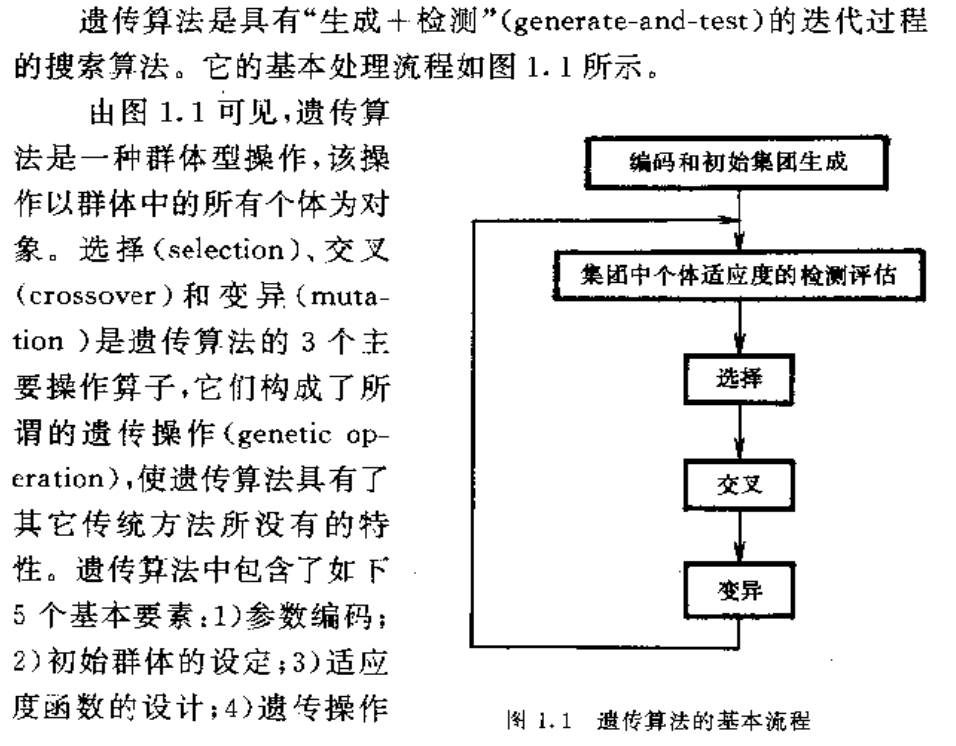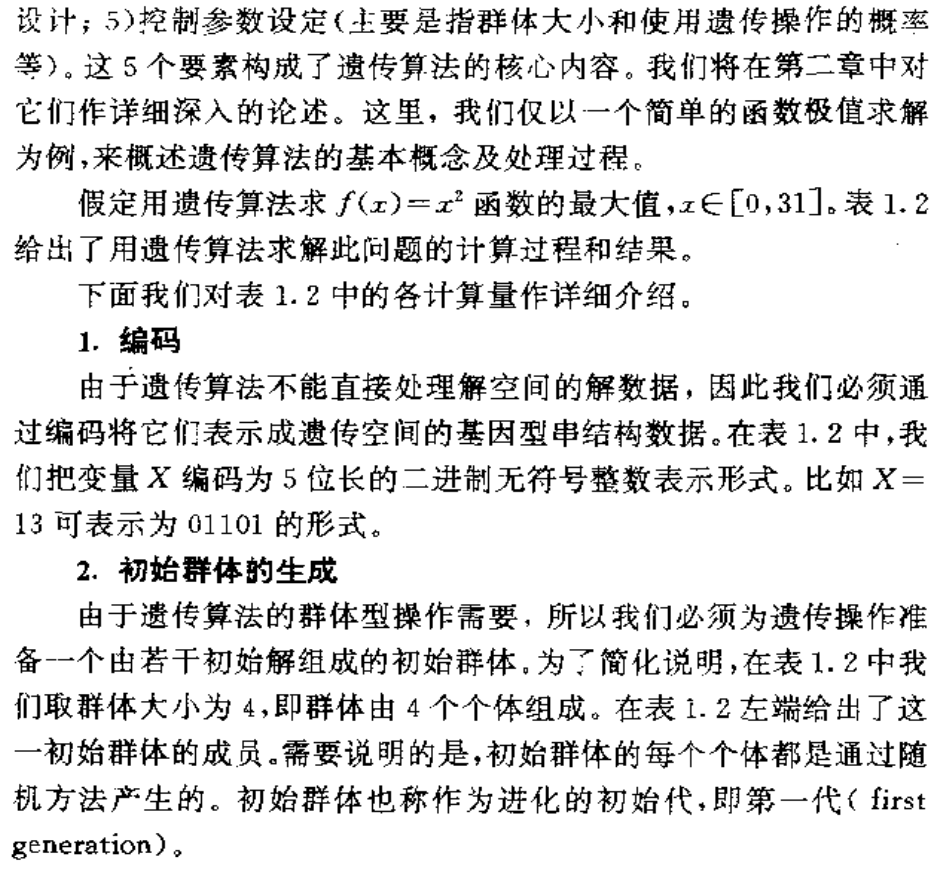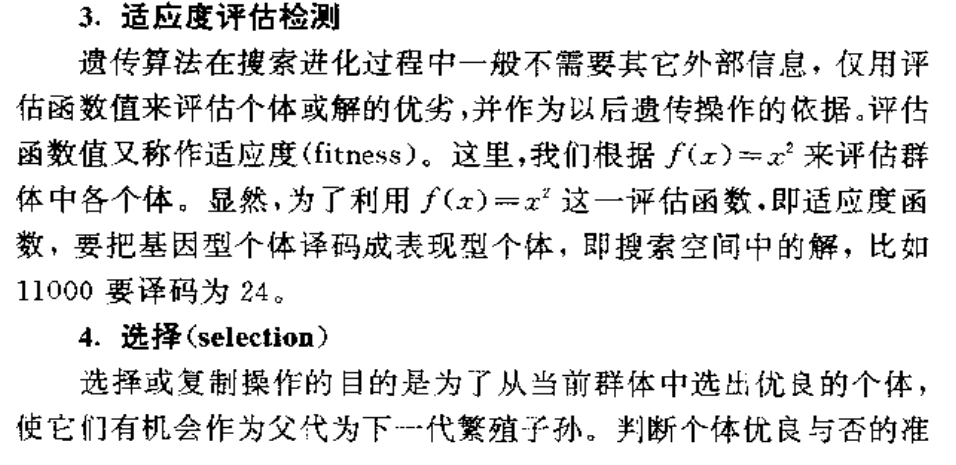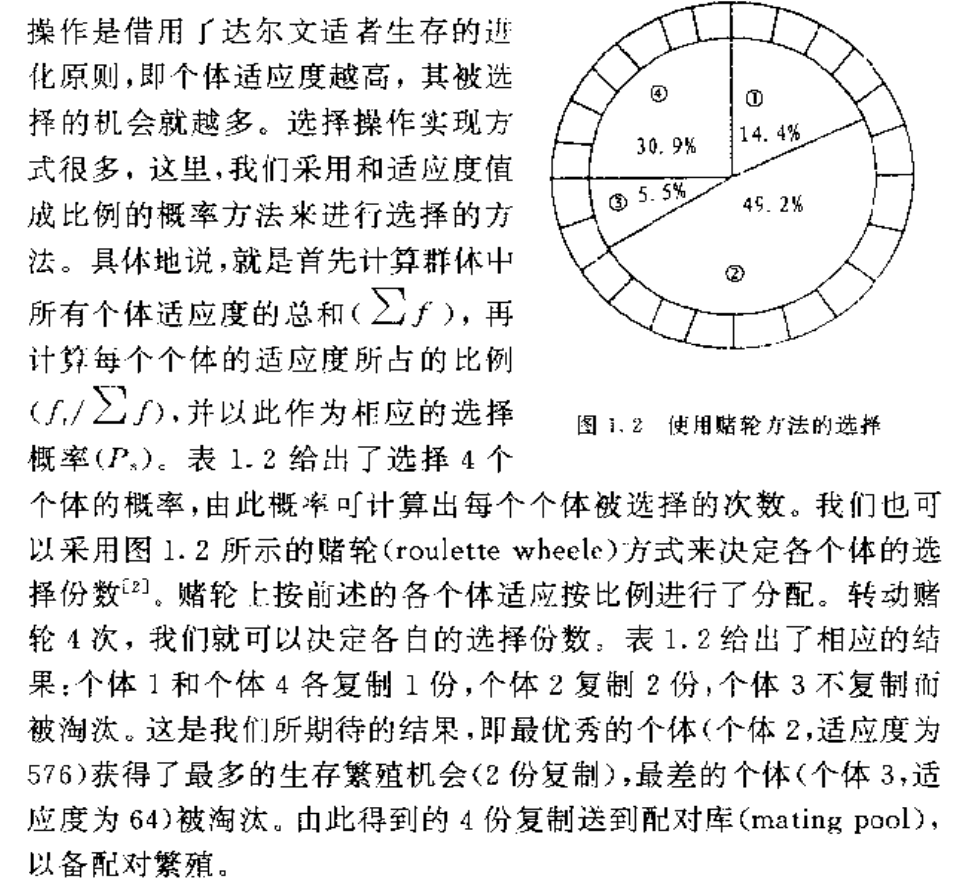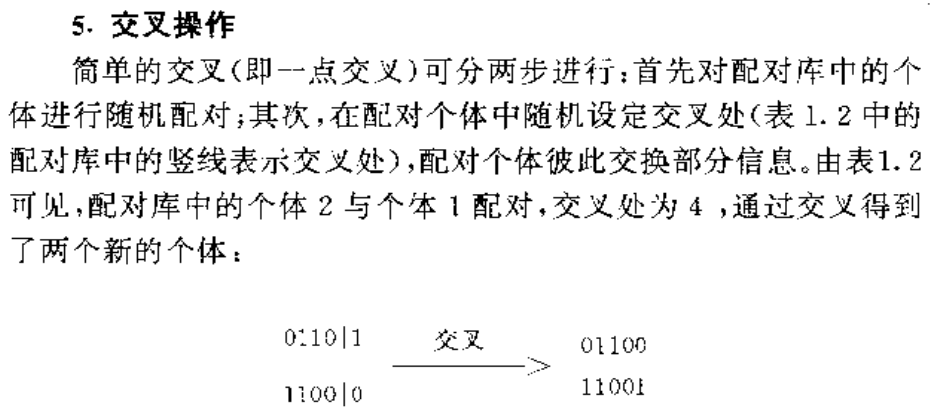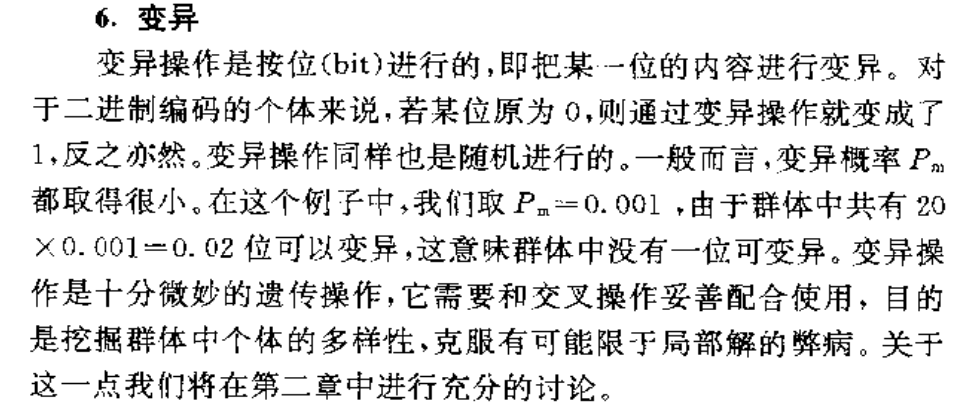《遗传算法及其应用》是在阅读GA过程中较好的一本算法基础方法介绍的书，想要从零开始详细的进行学习的同学们，这是很好的参考工具。
关于遗传算法，《遗传算法及其应用》一书给出了最为详尽的描述，书中也针对不同问题给出了基础的方法，例如组合优化问题中的函数优化、背包问题、货郎担问题和图论等等。

## 2.实例介绍

在上述内容的基础上，使用C++对函数优化问题进行求解，便于了解GA算法。这里使用的函数优化问题是无约束问题。
函数案例为：

min Z
Z=(1.5-x_1+x_1*x_2)^2+(2.25-x_1+x_1*(x_2)^2)^2+(2.625-x_1+x_1*(x_2)^3)^2
x->[-4.5,4.5]
最优解：Z(3,0.5)=0


2.1 编码
在示例函数当中，变量x_1和x_2均属于区间[-4.5,4.5]。使用二进制无符号整数进行编码，将变量约束空间映射到遗传空间。
为了更详细的表示遗传空间，以下使用8位二进制对变量进行编码，则编码空间整体位16位，其中前8位表示x_1的编码，其余表示x_2。
当然，这里使用5位编码也是可以的，只要能够包含约束区域即可。
2.2 解码
在2.1当中对变量进行了编码，这种编码从0000 0000开始，到1111 1111结束。
一般情况下0000 0000表示数值0，0000 00001表示数值1，以此类推。显然，无法将编码与约束进行一一对应。那么约束空间的负数和小数空间该如何进行表示呢？
假设约束空间的上限为b，下限为a，编码长度l，二进制编码转化为十进制值为x_t，实际值为x，则有：
x=a+(x_t *(b-a))/(2^l -1)
使用该方法对编码进行解码。解码方法如下：

void Decode(int X[person_number][node],int pers) {
int X_temp = 0;
for (int i = 0; i < 8; i++) {
X_temp += X[pers][i] * pow(2, 8 - 1 - i);
}
x_code[pers] = XMIN + XMIN + (X_temp*(XMAX - XMIN)) / (pow(2, 8) - 1);
X_temp = 0;
for (int i = 8; i < 16; i++) {
X_temp += X[pers][i] * pow(2, 16 - 1 - i);
}
x_code[pers] = XMIN + (X_temp*(XMAX - XMIN)) / (pow(2, 8) - 1);
}


为什么编码后，还需要进行解码呢？原因是将二进制码串转化为约束空间内的十进制值，便于适应度函数进行计算。
2.3 产生初始群体
对于初始群体，只需要使用rand()函数生成对应的0或1即可。
初始群体为：

void initial() {
srand((unsigned int)time(NULL));
for (int i = 0; i < person_number;i++) {
for (int j = 0; j < node;j++) {
X_code[i][j] = (rand() % (1 + 1));
}
}
}


2.4 轮盘赌选择
假设群体的适应性由一个转轮代表。
每一个染色体指定转轮中一个区域。区域的大小与该染色体的适应性分数成正比，适应性分数越高，它在转轮中对应的区域面积也越大。
为了选取一个染色体，要做的就是旋转这个轮子，当转轮停止时，指针停止在哪一区域上，就选中与它对应的那个染色体。
在这里，假设个体适应值为f_i；则个体的选择概率是f_i/sum(f_i)，概率越大，被选择的可能就越大，然后计算累积概率。
工作时，使用rand()函数生成0-1之间的小数，查看小数落在哪一累积概率区间，则选择该个体。
具体工作过程如下：

void Roulette() {
double fit_sum = 0;//适应值之和
for (int i = 0; i < person_number; i++) {
fit_temp[i] = Fitness(x_code, i);
fit_sum += fit_temp[i];
}
//注意:目标函数是取最小值，那么适应值越小，选择的概率越高
double fit_temp1[person_number];
double fit_sum1 = 0;//修改适应值之和
for (int i = 0; i < person_number; i++) {
fit_temp1[i]=fit_sum - fit_temp[i];//调整适应值为（和-适应值）
fit_sum1 += fit_temp1[i];
}
/*cout << "适应值：" << endl;
for (int i = 0; i < person_number; i++) {
cout<< fit_temp[i]<<" ";
}
cout << endl << "适应值和：" << fit_sum1 << endl;*/

for (int i = 0; i < person_number; i++) {

pro_select[i] = (int((fit_temp1[i] / fit_sum1)*1000000))/1000000.0;
}
pro_cumul = pro_select;
for (int i = 1; i < person_number; i++) {
pro_cumul[i] = pro_cumul[i-1]+pro_select[i];
}
pro_cumul[person_number-1] = 1.0;
}


2.5 选择
选择操作的本质为优胜略汰，选择原则是轮盘赌方法，选择的目的是将个体两两配对，放入交配池中，等待交配生成子代。
选择操作为：

void Selection() {
Roulette();//确定选择概率

for (int i = 0; i < person_number;i++) {
for (int j = 0; j < dimension;j++) {
per_cross[i][j] = -1;//初始化配对数组->满足交叉和变异的个体
}
}

//除了复制个体以外，两两配对：产生随机数->确定标号

//两两配对
srand((unsigned int)time(NULL));
for (int i = 0; i < person_number/2;i++) {
for (int j = 0; j < 2;j++) {
double temp = (rand() % (10000 + 1)) / 10000.0;
//查询下标
if (temp <= pro_cumul) {
per_cross[i][j] = 0;
}
else {
for (int k = 0; k < person_number - 1; k++) {
if (temp > pro_cumul[k]) {
if (temp <= pro_cumul[k + 1]) {
per_cross[i][j] = k + 1;
break;
}
}

}
}

}
}
/*cout << "交配池：" << endl;
for (int i = 0; i < person_number / 2; i++) {
for (int j = 0; j < 2; j++) {
cout << per_cross[i][j] << " ";
}
cout << endl;
}
cout << endl;*/
}


2.6 复制
复制的目的是保留最佳个体基因，将其保留到子代，有利于减少迭代次数。
复制操作为：

void Copy(double fit_temp[person_number]) {
//确定复制个体
double fit_temp1[person_number];//临时适应值数组
for (int i = 0; i < person_number; i++) {
fit_temp1[i] = fit_temp[i];
}
//适应值排序->由小到大
int fit_index[person_number];//适应值编号
for (int i = 0; i < person_number; i++) {
fit_index[i] = i;
}
for (int i = 1; i < person_number; i++) {
for (int j = 0; j < person_number - i; j++) {
if (fit_temp1[j + 1] < fit_temp1[j]) {
double temp1 = fit_temp1[j];
int temp2 = fit_index[j];
fit_temp1[j] = fit_temp1[j + 1];
fit_index[j] = fit_index[j + 1];
fit_temp1[j + 1] = temp1;
fit_index[j + 1] = temp2;
}
}
}
//确定复制个体->与目标函数有关，这里复制最小的个体
per_copy = fit_index;
fitness_temp = fit_temp1;
//cout << endl << "适应值："<< fit_temp1<<" "<<"最佳个体基因：" << per_copy << endl;
}


2.7 交叉
交叉是GA算法的重要操作。
在这里，使用二点交叉方法。二点交叉为：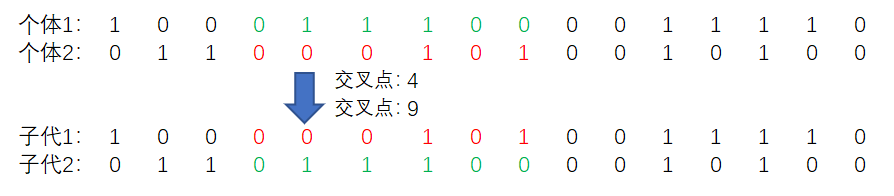若交配个体中含有最佳个体，则最佳个体直接复制到子代；交配对象的子代由最佳个体的交换部分加上自身未交换部分。
交叉操作为：

void Cross() {//二点交叉方法
//若复制个体与其他进行交叉，则复制为下一代，同时交叉一次（两种交叉结果中保留复制个体为主的子代）
//复制-》确定交叉点-》交叉
Copy(fit_temp);//确定复制个体
int X_code_tem[person_number][node];//临时二进制编码值
for (int i = 0; i < person_number;i++) {
for (int j = 0; j < node;j++) {
X_code_tem[i][j] = X_code[i][j];
}
}
srand((unsigned int)time(NULL));
int count = 0;//计数
for (int i = 0; i < person_number / 2;i++) {
double r1 = (rand() % (100 + 1)) / 100.0;
//cout <<endl<< "随机概率：" << r1 << endl;

int temp[node];//中转
for (int j = 0; j < node; j++) {
temp[j] = 0;
}
int X_code_tem1[node];
for (int m = 0; m < 2; m++) {
for (int n = 0; n < node; n++) {
X_code_tem1[m][n] = 0;
}
}
//cout << endl << "配对基因:"<<per_cross[i]<<" "<< per_cross[i] <<endl;
if (r1 < pc) {//可以进行交叉
//确定交叉点
int cross_section;//存放交叉点->小号在前
cross_section = rand() % (15 + 1);
cross_section = rand() % (15 + 1);
if ((cross_section == cross_section) && (cross_section == 0))
cross_section += 1;
if ((cross_section == cross_section) && (cross_section == 15))
cross_section -= 1;
if (cross_section > cross_section) {
swap(cross_section, cross_section);
}
//cout << endl << "交叉点：" << cross_section<<" "<< cross_section << endl;

//有最佳基因
if ((per_cross[i]==per_copy)&&(per_cross[i]!=per_copy)) {//有其中一个
for (int j = 0; j < node; j++) {
X_code_tem1[j] = X_code_tem[per_cross[i]][j];
X_code_tem1[j] = X_code_tem[per_cross[i]][j];
}

/*cout << endl;
for (int k = 0; k < 2; k++) {
for (int j = 0; j < node; j++) {
cout << X_code_tem1[k][j] << " ";
}
cout << endl;
}
cout << endl;*/

for (int j = cross_section; j < cross_section + 1; j++) {
X_code_tem1[j] = X_code_tem1[j];
}

for (int j = 0; j < node; j++) {
X_code[count][j] = X_code_tem1[j];
}
count++;
for (int j = 0; j < node; j++) {
X_code[count][j] = X_code_tem1[j];
}
count++;
}
else if ((per_cross[i] == per_copy) && (per_cross[i] != per_copy)) {//有其中一个
for (int j = 0; j < node; j++) {
X_code_tem1[j] = X_code_tem[per_cross[i]][j];
X_code_tem1[j] = X_code_tem[per_cross[i]][j];
}

/*cout << endl;
for (int k = 0; k < 2; k++) {
for (int j = 0; j < node; j++) {
cout << X_code_tem1[k][j] << " ";
}
cout << endl;
}
cout << endl;*/

for (int j = cross_section; j < cross_section + 1; j++) {
X_code_tem1[j] = X_code_tem1[j];
}

for (int j = 0; j < node; j++) {
X_code[count][j] = X_code_tem1[j];
}
count++;
for (int j = 0; j < node; j++) {
X_code[count][j] = X_code_tem1[j];
}
count++;
}
else {//没有最佳基因
for (int j = 0; j < node; j++) {
X_code_tem1[j] = X_code_tem[per_cross[i]][j];
X_code_tem1[j] = X_code_tem[per_cross[i]][j];
}

/*cout << endl;
for (int k = 0; k < 2; k++) {
for (int j = 0; j < node; j++) {
cout << X_code_tem1[k][j] << " ";
}
cout << endl;
}
cout << endl;*/

for (int j = cross_section; j < cross_section + 1; j++) {
temp[j] = X_code_tem1[j];
}
for (int j = cross_section; j < cross_section + 1; j++) {
X_code_tem1[j] = X_code_tem1[j];
}
for (int j = cross_section; j < cross_section + 1; j++) {
X_code_tem1[j] = temp[j];
}

for (int j = 0; j < node; j++) {
X_code[count][j] = X_code_tem1[j];
}
count++;
for (int j = 0; j < node; j++) {
X_code[count][j] = X_code_tem1[j];
}
count++;
}

}
else {//不交叉，直接复制
for (int j = 0; j < node; j++) {
X_code_tem1[j] = X_code_tem[per_cross[i]][j];
X_code_tem1[j] = X_code_tem[per_cross[i]][j];
}

/*cout << endl;
for (int k = 0; k < 2; k++) {
for (int j = 0; j < node; j++) {
cout << X_code_tem1[k][j] << " ";
}
cout << endl;
}
cout << endl;*/

for (int j = 0; j < node; j++) {
X_code[count][j] = X_code_tem1[j];
}
count++;
for (int j = 0; j < node; j++) {
X_code[count][j] = X_code_tem1[j];
}
count++;

}
}

/*cout << "交叉个体：" << endl;
for (int i = 0; i < person_number; i++) {
for (int j = 0; j < node; j++) {
cout << X_code[i][j] << " ";
}
cout << endl;
}
cout << endl;*/
}


## 3.约束问题优化

对于带约束的函数优化问题，可以参照PSO算法求解的内容，使用罚函数的方法进行约束处理，转变为无约束问题求解。

此外，遗传算法的高级实现方式以及并行算法，是对求解过程的改进，与SGA方法相比，改进的方法求解速度更快，准确度更高，也能避免局部最优的发生。

以上内容，如有引用不当，将立即修改。

欢迎各位同学进行交流！

展开全文• 一种新的求解复杂函数优化问题的并行粒子群算法.pdf
• 基于MATLAB的遗传算法函数优化问题求解实验报告 一、实验要求： ...绘出函数图像，并给出matlab工具箱函数命令下应用遗传算法求解优化问题的结果和结果分析。 (2) 优化问题： Max f(x,y), s. t. -3

## 基于MATLAB的遗传算法函数优化问题求解实验报告

### 一、实验要求：

掌握matlab遗传算法工具箱的函数命令实现函数优化问题的方法和图形用 户界面下求解优化问题的方法。

1. 利用matlab工具箱函数命令实现函数的优化：

已知：

（1）f(x)=x+10sin(5x)+7cos(4x)

优化问题：

Max f(x), s. t. 15£ x£25

绘出函数图像，并给出matlab工具箱函数命令下应用遗传算法求解优化问题的结果和结果分析。

(2)优化问题：

Max f(x,y), s. t. -3£x£3,-3£y£3

绘出函数图像，并给出matlab工具箱函数命令下应用遗传算法求解优化问题的结果和结果分析。

2、图形用户界面下应用遗传算法求解优化问题:

已知：

（1）f(x)=x+10sin(5x)+7cos(4x)

优化问题：

Max f(x), s. t. 15£ x£25

给出图形用户界面下应用遗传算法求解优化问题的结果和结果分析。

(2)优化问题：

Max f(x,y), s. t. -3£x£3,-3£y£3

给出图形用户界面下应用遗传算法求解优化问题的结果和结果分析。

### 二、实验内容

#### 2.1 利用matlab工具箱函数命令实现函数的优化

语法规则：

x = ga(fitnessfcn,nvars)x = ga(fitnessfcn,nvars,A,b)x = ga(fitnessfcn,nvars,A,b,Aeq,beq)x = ga(fitnessfcn,nvars,A,b,Aeq,beq,LB,UB)%
其中fitnessfc为函数的句柄或者为匿名函数
nvars,表示自变量个个数（例如自变量为向量X,nvars代表X中的元素个数）
A，b就是表达式A*X<=b;
Aeq:表示线性等式约束矩阵，若是没有等式约束就写为[];
Beq:表示线性等式约束的个数Beq=length(nvars);

##### 2.1.1 f(x)=x+10sin(5x)+7cos(4x)
###### 2.1.1.1 函数图像：
x = 0:0.01:25;
y = x+10*sin(5*x)+7*cos(4*x);
plot(x,y)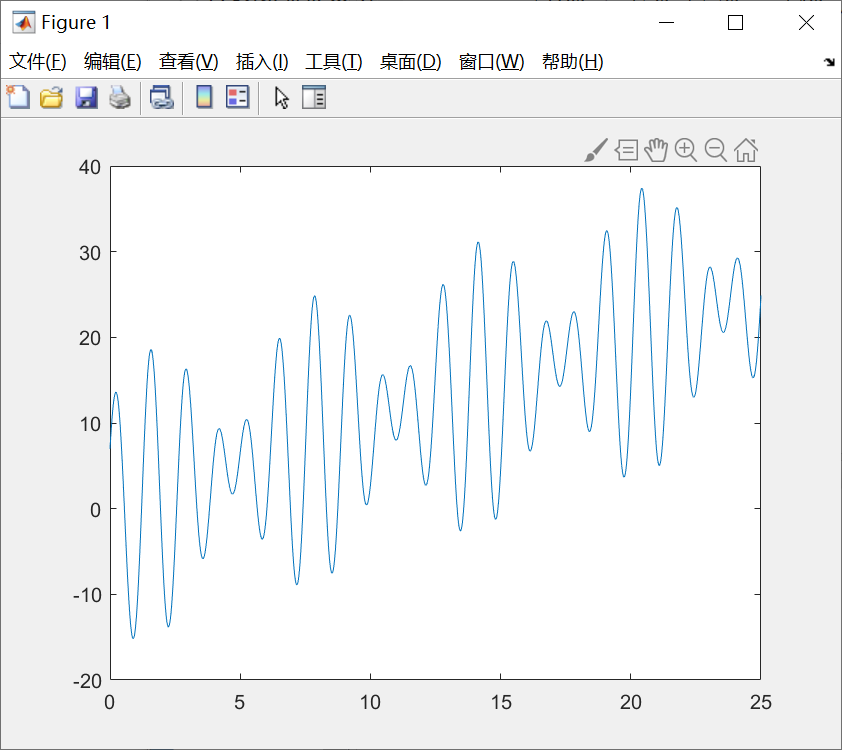###### 2.1.1.2 适应度函数：
function y = f1(x)
y = -(x+10*sin(5*x)+7*cos(4*x));
end

###### 2.1.1.3 使用ga函数求解最大值：
x = 0:0.01:20;
y = x+10*sin(5*x)+7*cos(4*x);
plot(x,y)
hold on
OF = @f1;
nvars = 1;
LB = -20;
UB = 20;
[x,fval] = ga(OF,nvars,[],[],[],[],LB,UB)
plot(x,-fval,'*')

##### 2.1.2###### 2.1.2.1 函数图像：
t=-3:.01:3;
[x,y]=meshgrid(t);%形成格点矩阵
z=-f2(x,y);
figure(1)
mesh(x,y,z);
axis([-3 3 -3 3 -10 10]);
title('z = (3.*((1-x).^2).*exp(-x.^2-(y+1).^2)-10.*(x./5-x.^3-y.^5).*exp(-x.^2-y.^2)-(1/3).*exp(-(x+1).^2-y.^2)); mesh')
colormap cool%cool是一种配色方案，还有其他方案如winter，summer····见help colormap
colorbar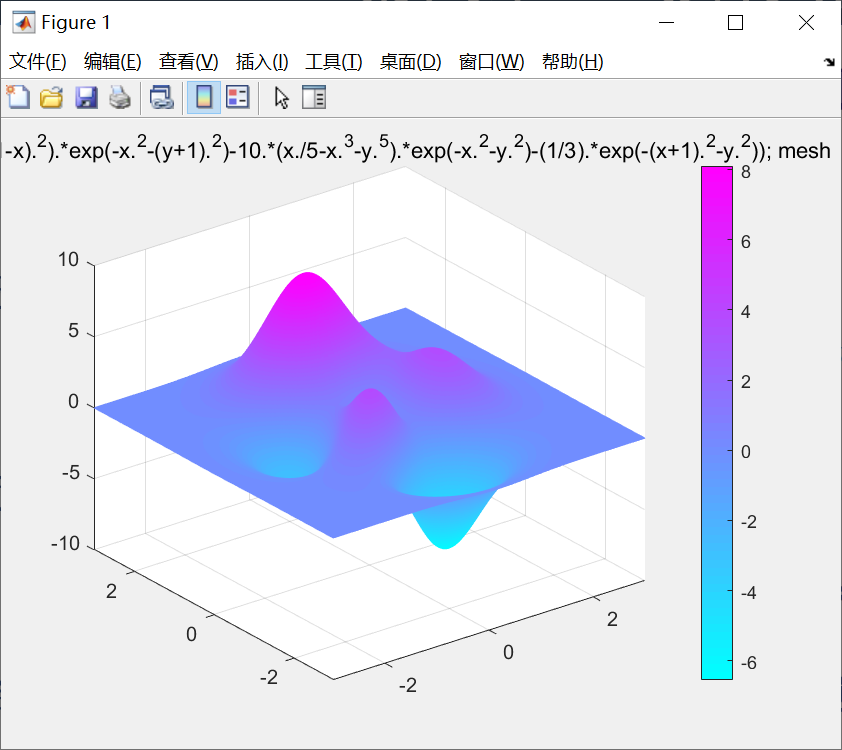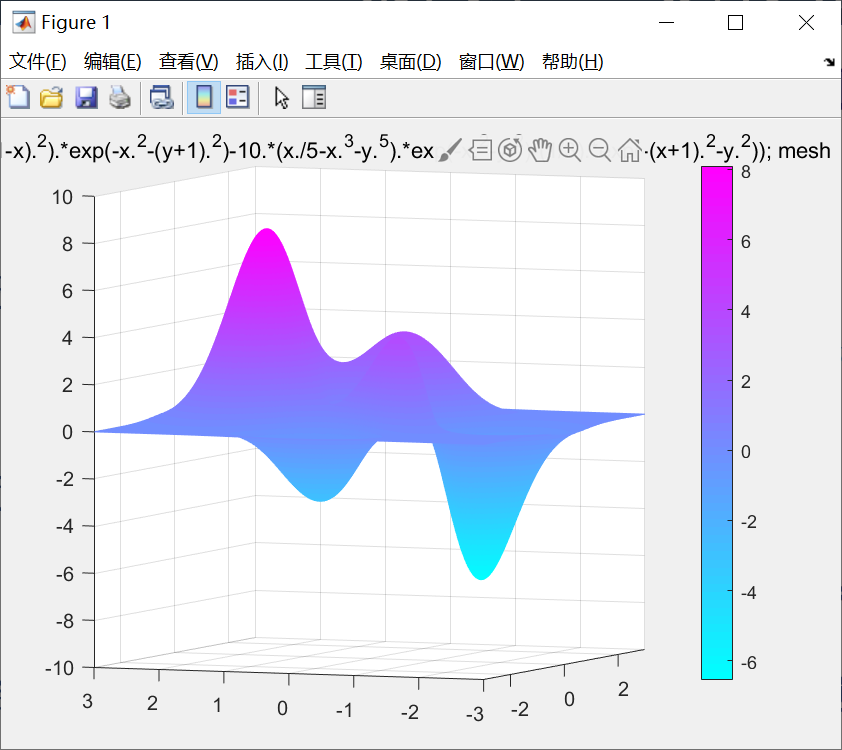###### 2.1.2.2 适应度函数：
function z = f2(x)
z = -(3*((1-x(1))^2)*exp(-x(1)^2-(x(2)+1)^2)-10*(x(1)/5-x(1)^3-x(2)^5).*exp(-x(1)^2-x(2)^2)-(1/3)*exp(-(x(1)+1)^2-x(2)^2));
end

###### 2.1.2.3 使用ga函数求解最大值：
f = @(x) 3*((1-x(1))^2)*exp(-x(1)^2-(x(2)+1)^2)-10*(x(1)/5-x(1)^3-x(2)^5)*exp(-x(1)^2-x(2)^2)-(1/3)*exp(-(x(1)+1)^2-x(2)^2);
nvars = 2;
lb = [-3; -3]; % x y的下限
ub = [3; 3] ; % x y的上限
[x,fval] = ga(f,nvars,[],[],[],[],lb,ub)



#### 2.2 图形用户界面下应用遗传算法求解优化问题:

##### 2.2.1 f(x)=x+10sin(5x)+7cos(4x)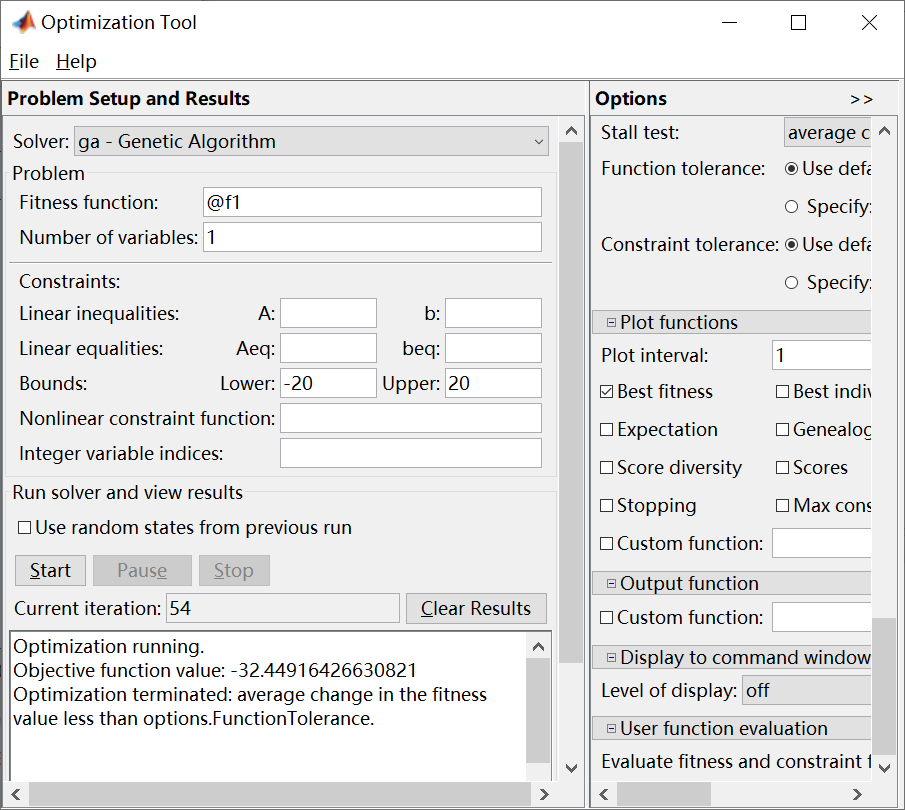##### 2.2.2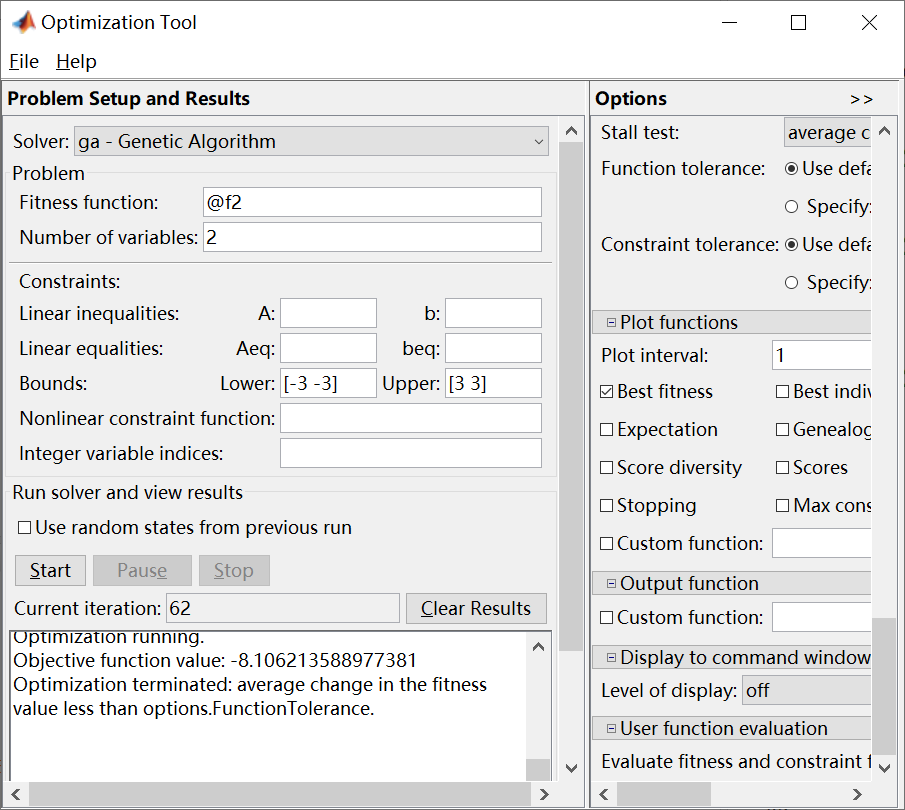### 三、运行结果

#### 3.1 利用matlab工具箱函数命令实现函数的优化

##### 3.1.1 f(x)=x+10sin(5x)+7cos(4x)
###### 运行结果：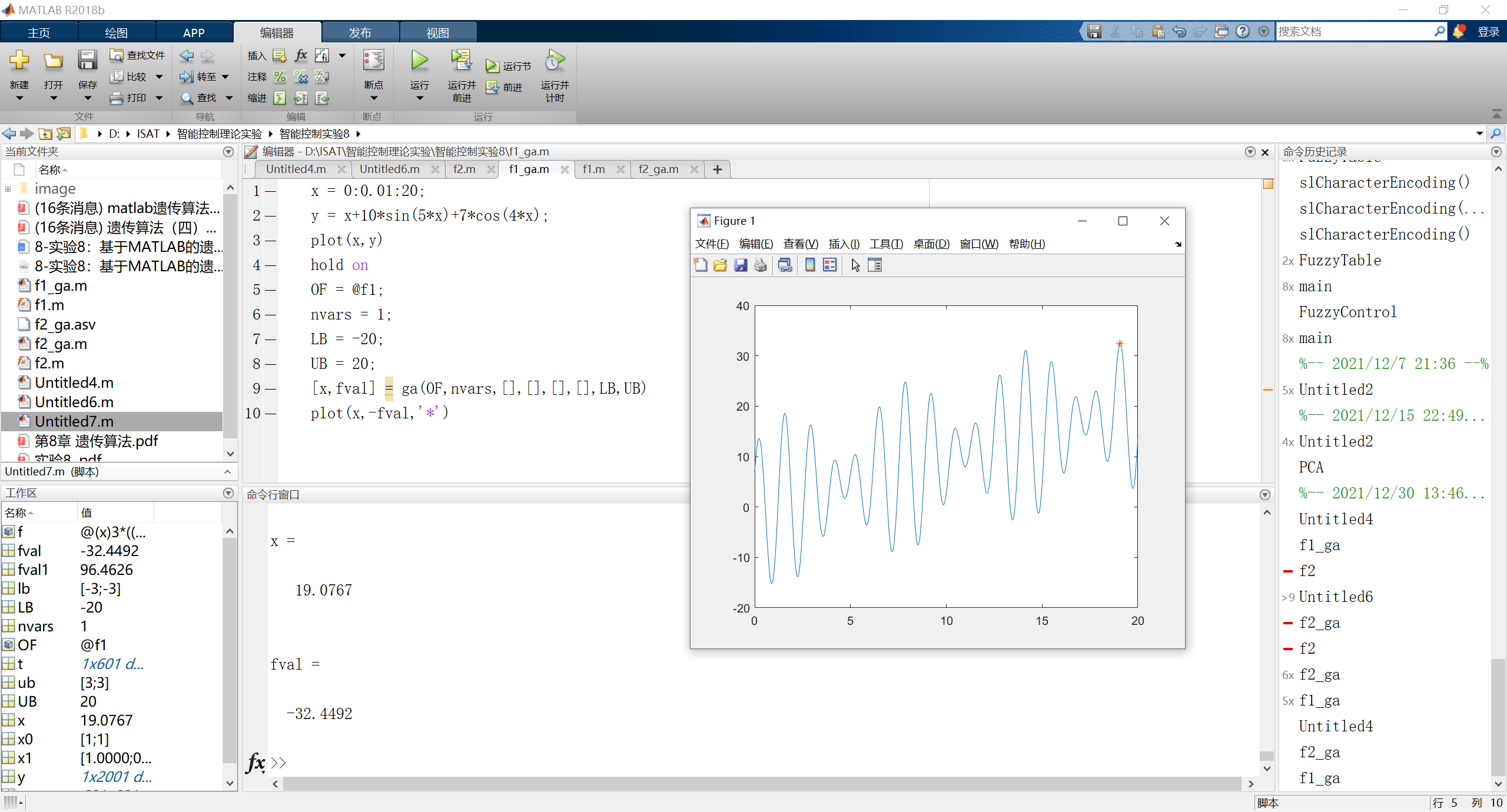###### 结果分析：

遗传算法很快得到了函数的最大值，但是并不是每次运行都能得到最优解，有时会陷入局部最大值中。

##### 3.1.2###### 运行结果：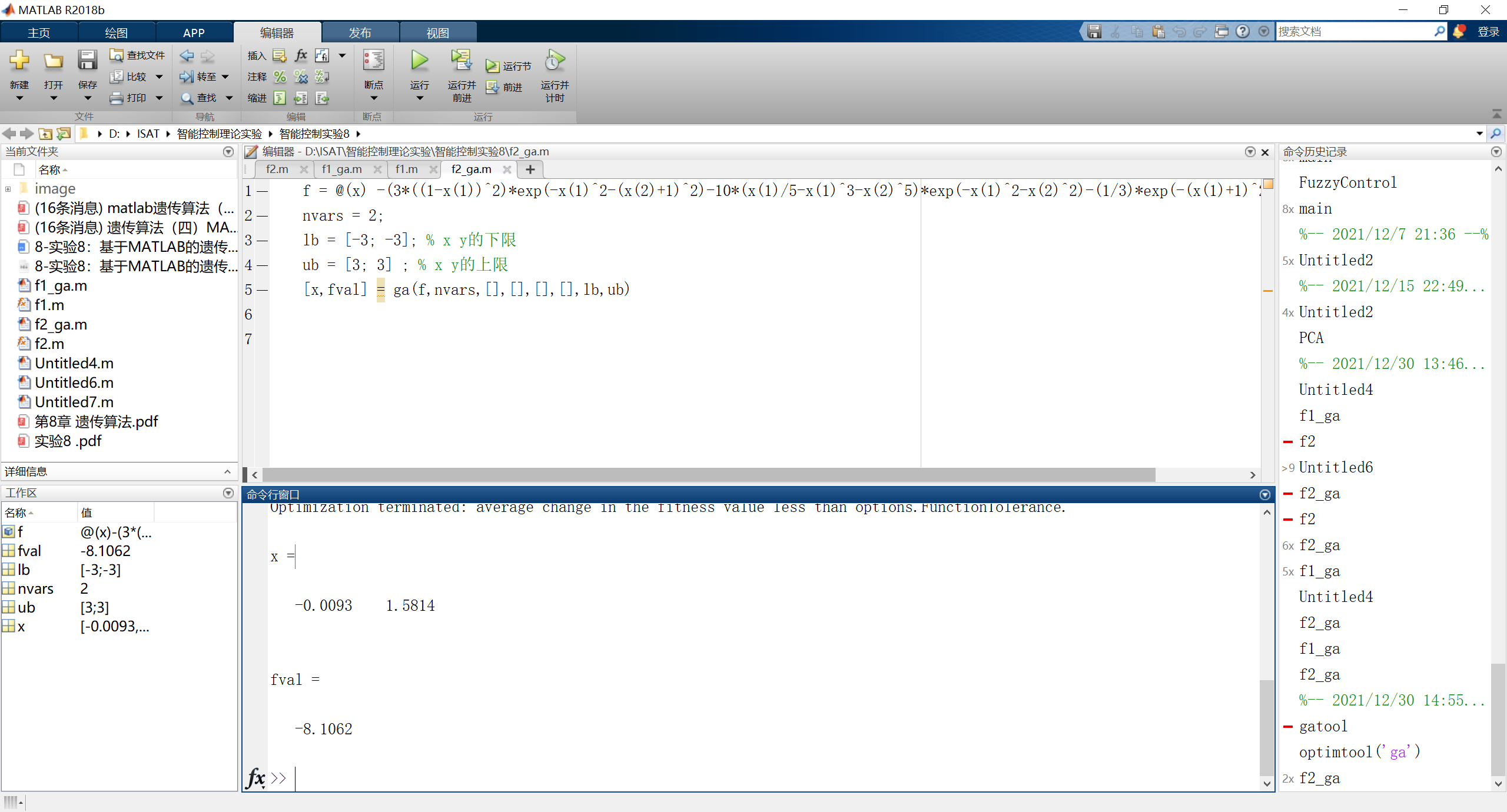###### 结果分析：

根据图像观察，该二元函数的最大值大概在6~8之间，使用ga函数求解的最大值8.1062，符合要求。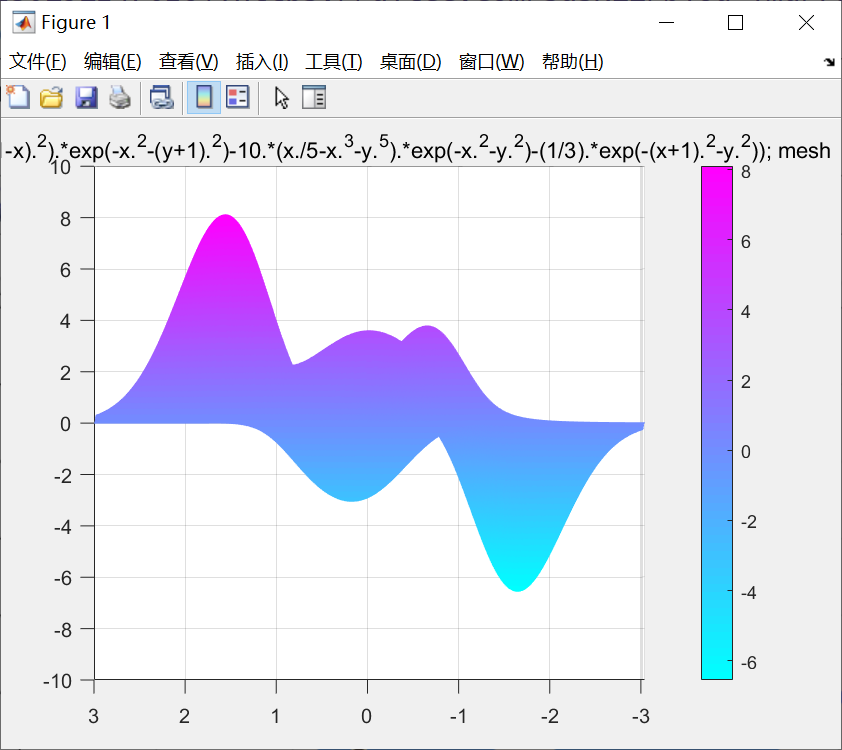#### 3.2 图形用户界面下应用遗传算法求解优化问题:

##### 3.2.1 f(x)=x+10sin(5x)+7cos(4x)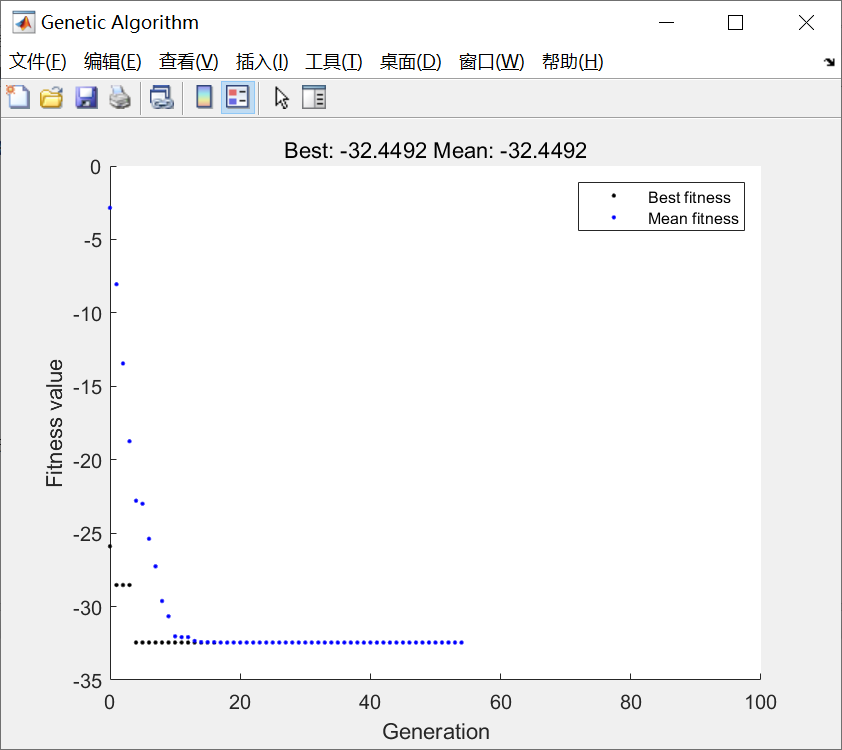##### 3.1.2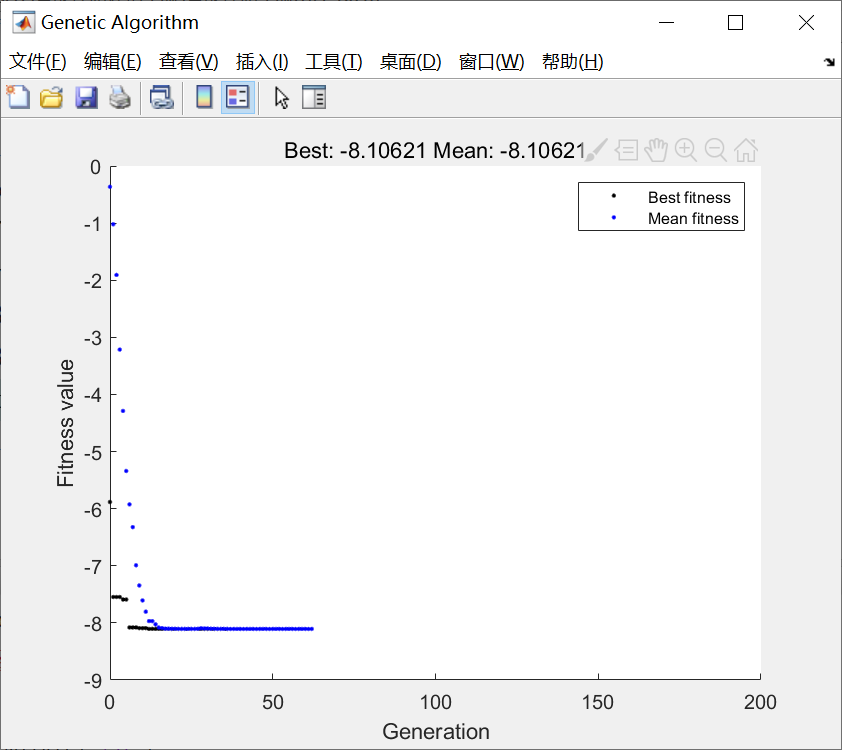### 四、总结：

（1）GA是对问题参数的编码（染色体）进行操作，而不是参数本身。
（2）GA计算简单，便于计算机编程，功能强。
（3）GA是从问题解的串集开始搜索，而不是从单个解开始，更有利于搜索到全局最优解。
（4）GA使用对象函数值（即适应值）这一信息进行搜索，而不需导数等其它信息。
（5）GA的复制、交叉、变异这三个算子都是由概率决定的，而非确定性的。
（6）GA算法具有隐含的并行性，因而可通过大规模并行计算来提高计算速度。
（7）GA更适合大规模复杂问题的优化，但解决简单问题效率并不高。

展开全文matlab 开发语言
• 基于Spark的并行遗传算法求解多峰函数极值.pdfhadoop
• 提出了一种求解多峰函数优化问题的免疫量子进化算法，该算法依据小生境机制将量子表达的初始种群划分为子群组，再对每个子群组利用免疫特性的局域搜索能力包括抗体的克隆选择、记忆细胞产生、免疫细胞交叉变异、抗体...
• 介绍了一种利用量子行为粒子群算法（QPSO）求解多峰函数优化问题的方法。为此，在QPSO中引进一种物种形成策略，该方法根据群体微粒的相似度并行地分成子群体。每个子群体是围绕一个群体种子而建立的。对每个子群体...
• 针对传统演化算法求解函数优化，特别是多峰函数优化问题中出现的早熟现象以及演化后期收敛速度慢等问题，提出了一种新的反序小生境演化算法。该算法采用小生境反序交叉算子，以进一步增强局部寻优的能力；引入一种...
• 复杂函数的全局最优化问题是在求解各种复杂工程与科学计算问题中提炼出来的亟待解决的计算...基于均匀设计与Powell算法的全局最优化并行算法具有寻优能力强，时间开销与问题因素个数的平方和布点数成线性复杂度，空间开均匀设计
• 复杂函数的全局最优化问题是在求解...基于均匀设计与Powell算法的全局最优化并行算法具有寻优能力强,时间开销与问题因素个数的平方和布点数成线性复杂度,空间开销与因素个数和布点数成线性复杂度,并行效率好的特点。
• 针对处理大量数据和求解大规模复杂问题时粒子群...通过对多个基准优化测试函数求解证明, 相对于基于CPU的串行计算方法, 在求解收敛性一致的前提下, 基于CUDA架构的并行PSO求解方法可以取得高达90倍的计算加速比。
• 完整代码已上传我的资源：【单目标优化求解】基于matlab粒子群算法求解非线性目标函数最小值问题【含Matlab源码 1573期】 备注： 订阅紫极神光博客付费专栏，可免费获得1份代码（有效期为订阅日起，三天内有效）； ...matlab 开发语言
• 针对处理大量数据和求解大规模复杂问题时粒子群优化(PSO)算法计算时间过长的问题, 进行了在显卡(GPU)上实现细粒度并行粒子群算法的研究。通过对传统PSO算法的分析, 结合目前被广泛使用的基于GPU的并行计算技术, 设计...
• 演化计算又称为进化算法、进化计算，是一种元启发式方法。搜索过程是从一个初始解的集合（称为初始种群）开始的，种群中的每一个解都沿着一定的轨迹搜索，每前进一步称为种群的进化，得到的解集称为种群的一代。这样...数据挖掘
• 1 简介 ...蚂蚁算法本质上是一种用于解决组合优化问题的概率型算法。它的仿生学基础源自于蚂蚁觅食过程中的路径搜索行为。该算法具有并行计算、启发式搜索和信息正反馈的特点。蚂蚁算法被大量应用于TCmatlab 开发语言
• 为了提高工程优化问题的寻...函数优化以及分包商选择等组合优化问题可利用该算法进行有效求解。仿真实验结果表明:对于相同的优化问题,改进的并行混沌优化算法可以求得更好的优化解,从而证明该方法具有良好的寻优性能。
• 模拟退火法和蚁群算法求解多元函数极值问题 目标函数： 算法概述 模拟退火算法 模拟退火算法(Simulated Annealing，简称SA)的思想最早是由Metropolis等提出的。其出发点是基于物理中固体物质的退火过程与一般的组合...
• 粒子群算法是一种并行算法。 2） 什么？ 看不懂？ 我来通俗解释： 粒子群算法是生物学家研究鸟类捕食创造的，把一只鸟比作成一个粒子，设想一个有20只秃鹫（粒子）的群体吧，秃鹫相互独立具有个体特征但又相互...matlab
• 1、遗传算法（GA）介绍 ...在求解较为复杂的组合优化问题时,相对一些常规的优化算法,通常能够较快地获得较好的优化结果。遗传算法已被人们广泛地应用于组合优化、机器学习、信号处理、自适应控制和人工生python
• 针对基于可行性规则求解约束优化问题易陷入局部、master-slave协同进化模型...典型函数测试表明，MSMHCO算法和同类算法相比,收敛速度更快,求解精度更高。丁烯烷化过程的约束优化实例也进一步证明了MSMHCO算法的有效性。模拟退火
• 最直接，最易于理解的设计方法，发掘和利用现有串行算法中的并行性，直接将串行算法改造为并行算法。 Case 1：快速排序 ​ 快速排序的串行算法思想为随机选取主元进行划分，之后递归排序。直接并行化思路即每次划分...排序
• 利用遗传算法求Rosenbrock(香蕉函数)函数的极大值。其中香蕉函数表达式如下： 遗传算法是利用孟德尔的生物遗传学和达尔文的进化论思想的一种智能计算方法。我觉着与其称之为一种算法，倒不如称之为是一种思想。 ...人工智能 极值 MATLAB
• 例：求下述二元函数的最大值：(1) 个体编码遗传算法的运算对象是表示个体的符号串，所以必须把变量 x1, x2 编码为一种符号串。本题中，用无符号二进制整数来表示。因 x1, x2 为 0 ~ 7之间的整数，所以分别用3位无...
• 依据各级缓存容量，将CPU主存中种群个体和蚂蚁个体数据划分存储到一级、二级和三级缓存中，以减少并行计算过程中数据在各级存储之间的传输开销，在CPU与GPU之间采取异步传送和不完全传送数据、GPU多个内核函数异步...
• 优化模拟退火算法 作为现代算法的一种，模拟退火算法是一种用降低搜索的覆盖面积来提高运算速度的算法，适用于解决各种优化类问题。它利用了物理学中一个常见的原理：当物体具有一定的温度时，假设它内部含有的能量...
• 算法专为评估成本高昂的黑盒函数的全局多目标优化而设计。 例如，该算法已应用于生命周期评估（LCA）和化学过程仿真成本的同时优化。 但是，该算法也可以应用于其他黑盒函数，例如 CFD 模拟。 它基于贝叶斯...matlab
• 粒子群优化(PSO)是一种基于群体智能的数值优化算法，由社会心理学家James Kennedy和电气工程师Russell Eberhart于1995年提出。自PSO诞生以来，它在许多方面都得到了改进，这一部分将介绍基本的粒子群优化算法原理和...matlab 机器学习
• 将该问题建模为一个多约束条件的组合优化问题，根据遗传算法特别适合求解大规模组合优化问题的特点，设计了一种粗粒度并行遗传算法来对此优化问题进行求解。在消息传递类并行软件开发环境提供的基于消息传递的并行......